# ICSE Class 8 Maths Selina Solutions Chapter 1 Rational Numbers

ICSE Class 8 Maths Chapter 1 – Rational Numbers, covers topics such as properties of rational numbers, representation of rational numbers, word problem and more. For a subject like Maths, firstly students should go through the chapter and by hard the formulas thoroughly. After doing so, they should try answering the questions given in the Seline textbook. Once done with the solving, they should evaluate their answers by comparing it with the ICSE Selina Class 8 Maths Chapter 1 – Rational Numbers Solution by BYJU’S.

Rational Number – A number that can be made by dividing two integers.
Representation of Rational Numbers on Number Line – Representation on number line depends upon the type of rational fraction is to be represented on the line. Positive rational numbers are always represented on the right side of the zero on the number line. While negative rational numbers are always represented on the left side of zero on the number line.
By solving these solutions the students will gain the confidence to solve the different types of questions that might come in the examination. The ICSE Selina Class 8 Maths Chapter 1 – Rational Numbers can be downloaded from the links below.

## Download ICSE Class 8 Maths Selina Solutions PDF for Chapter 1:-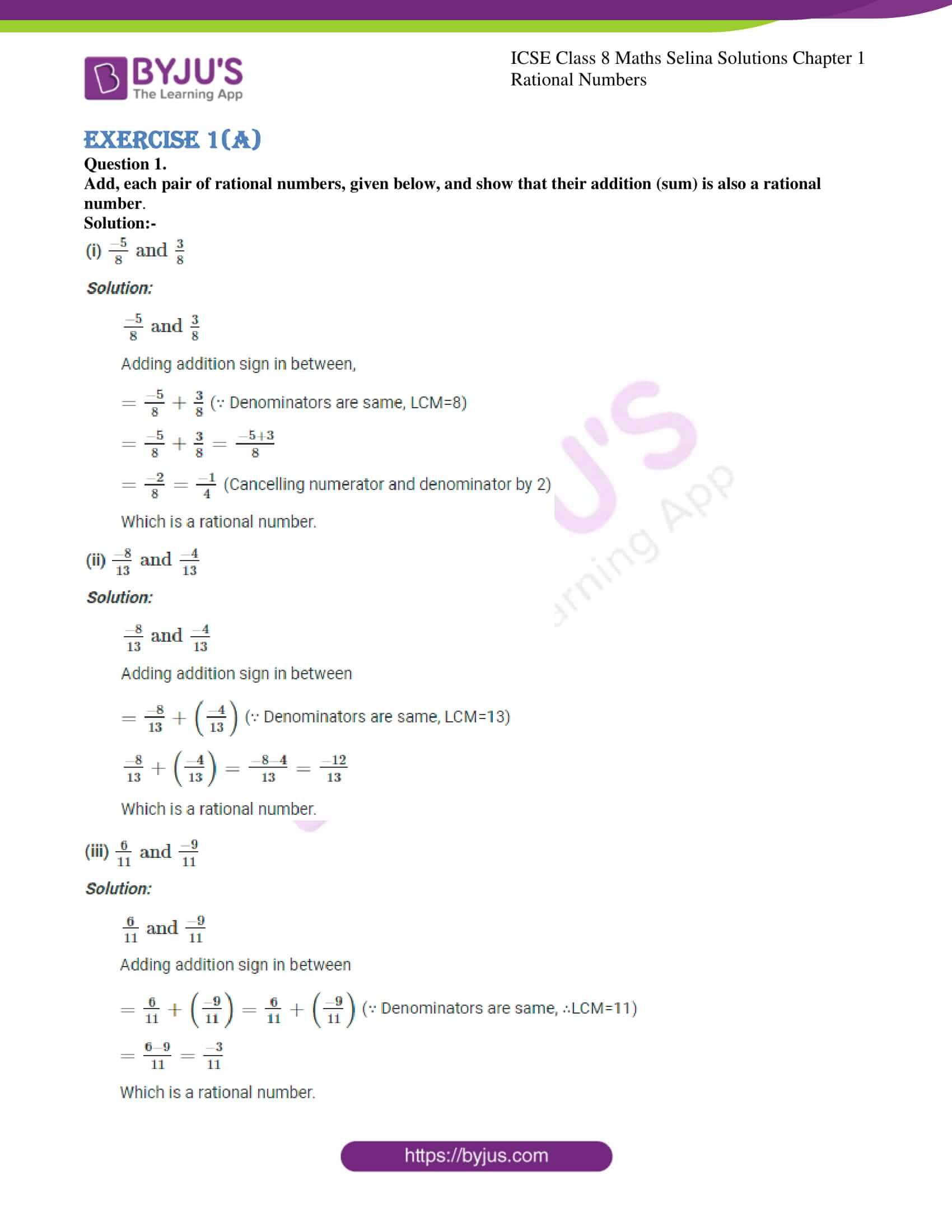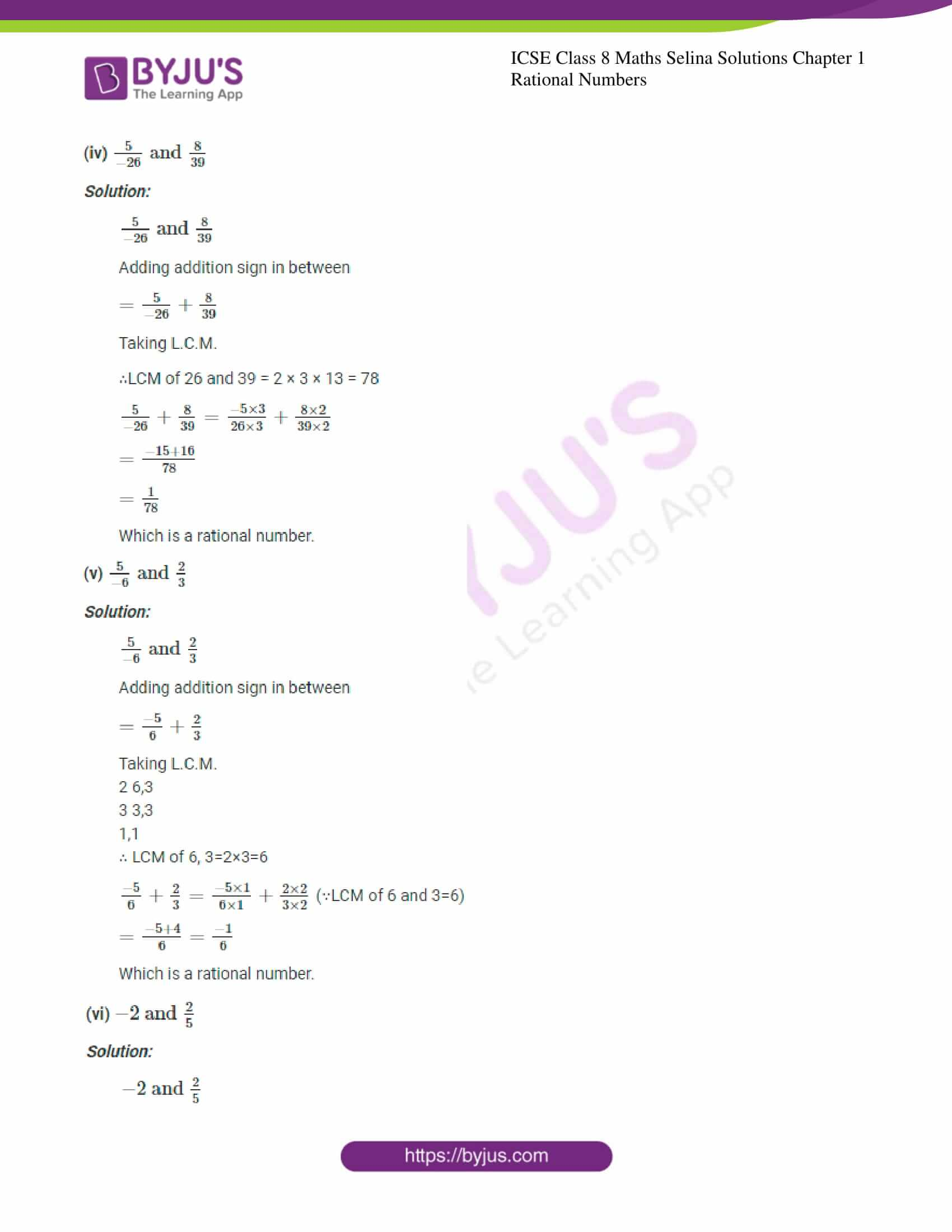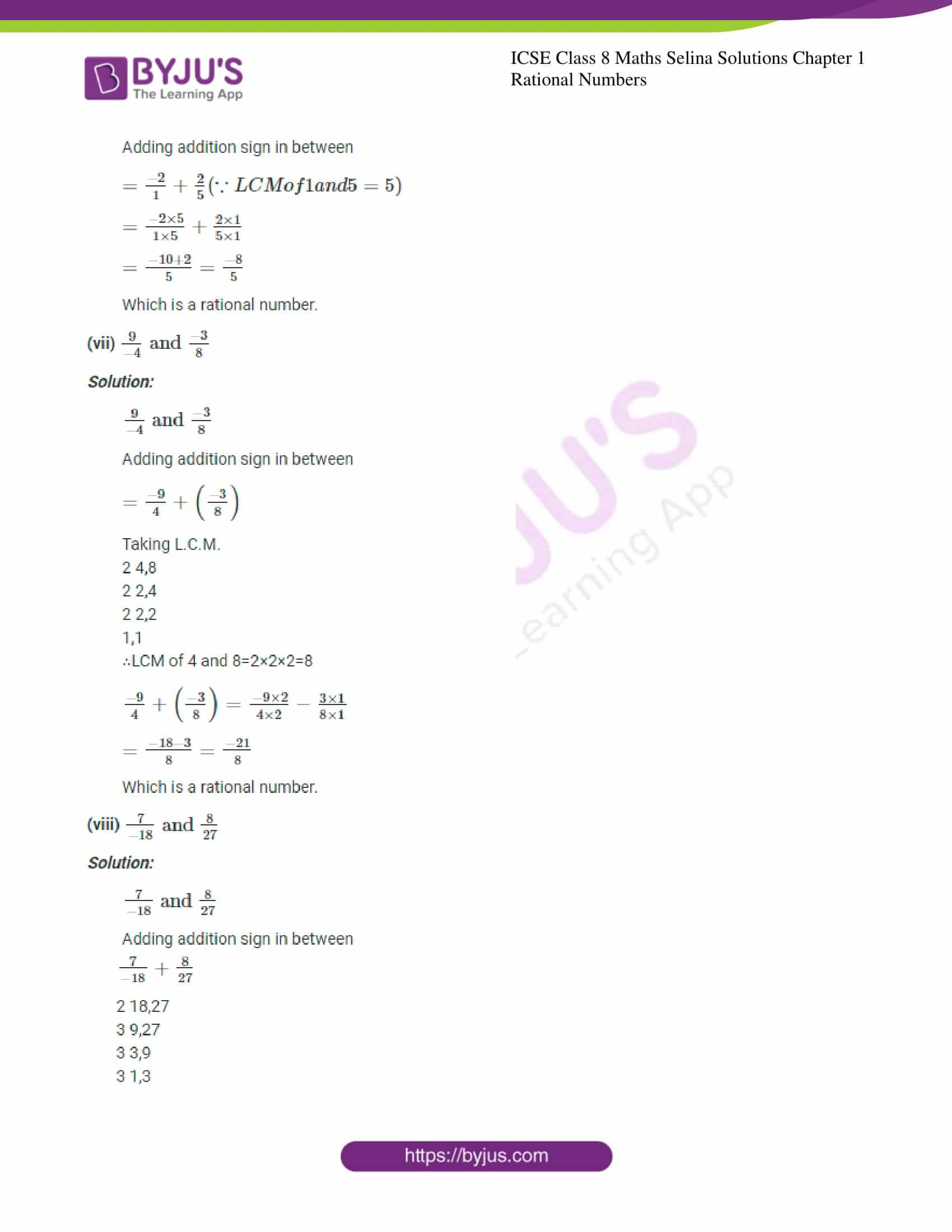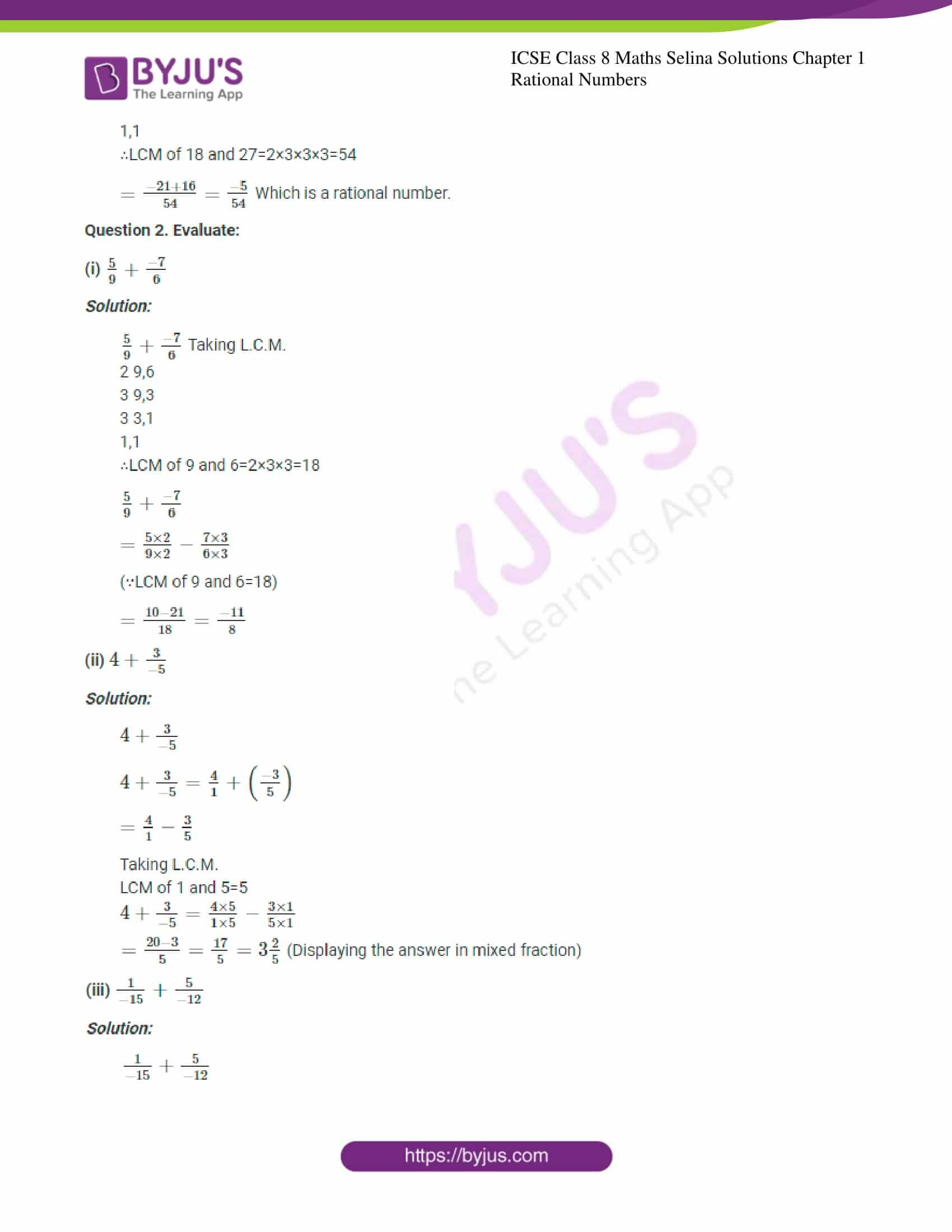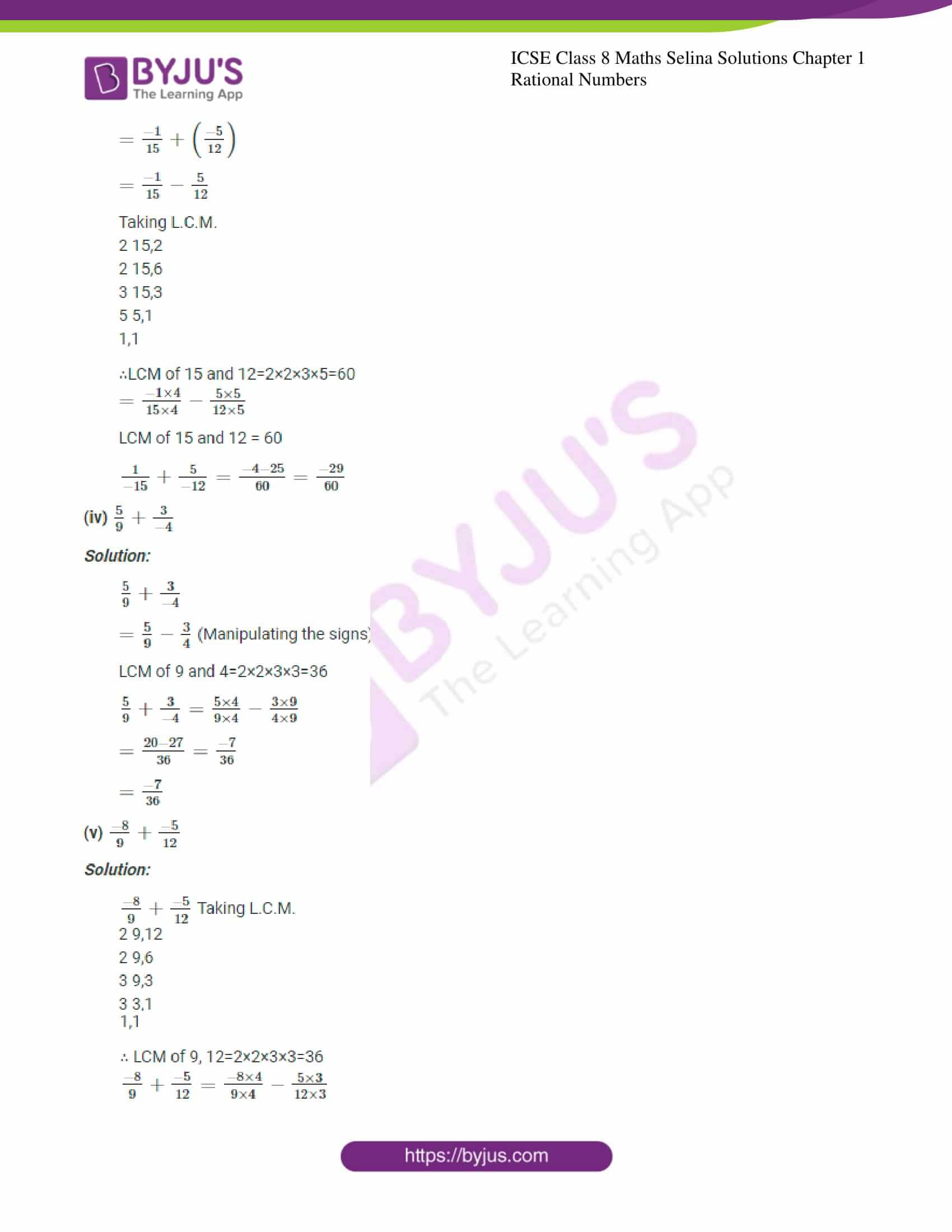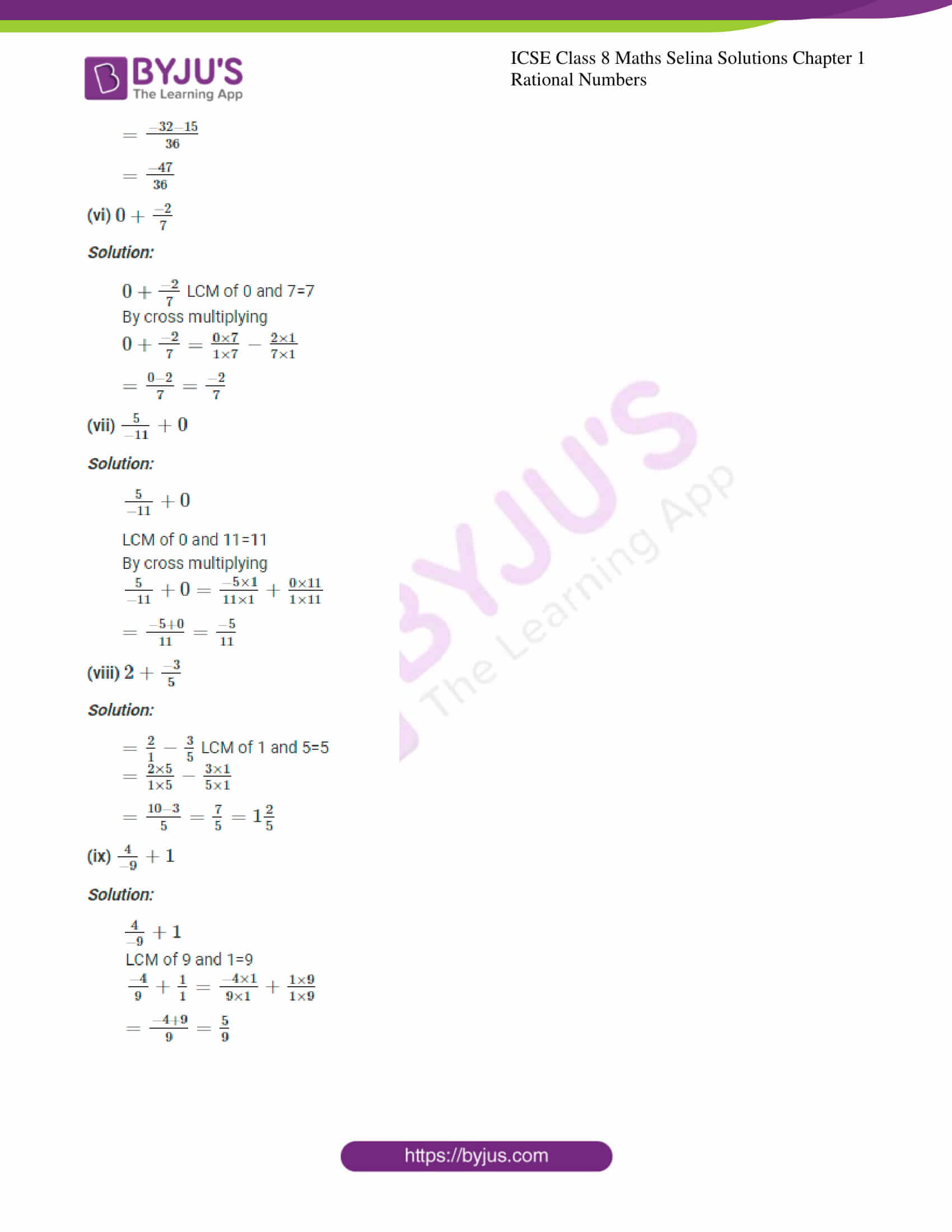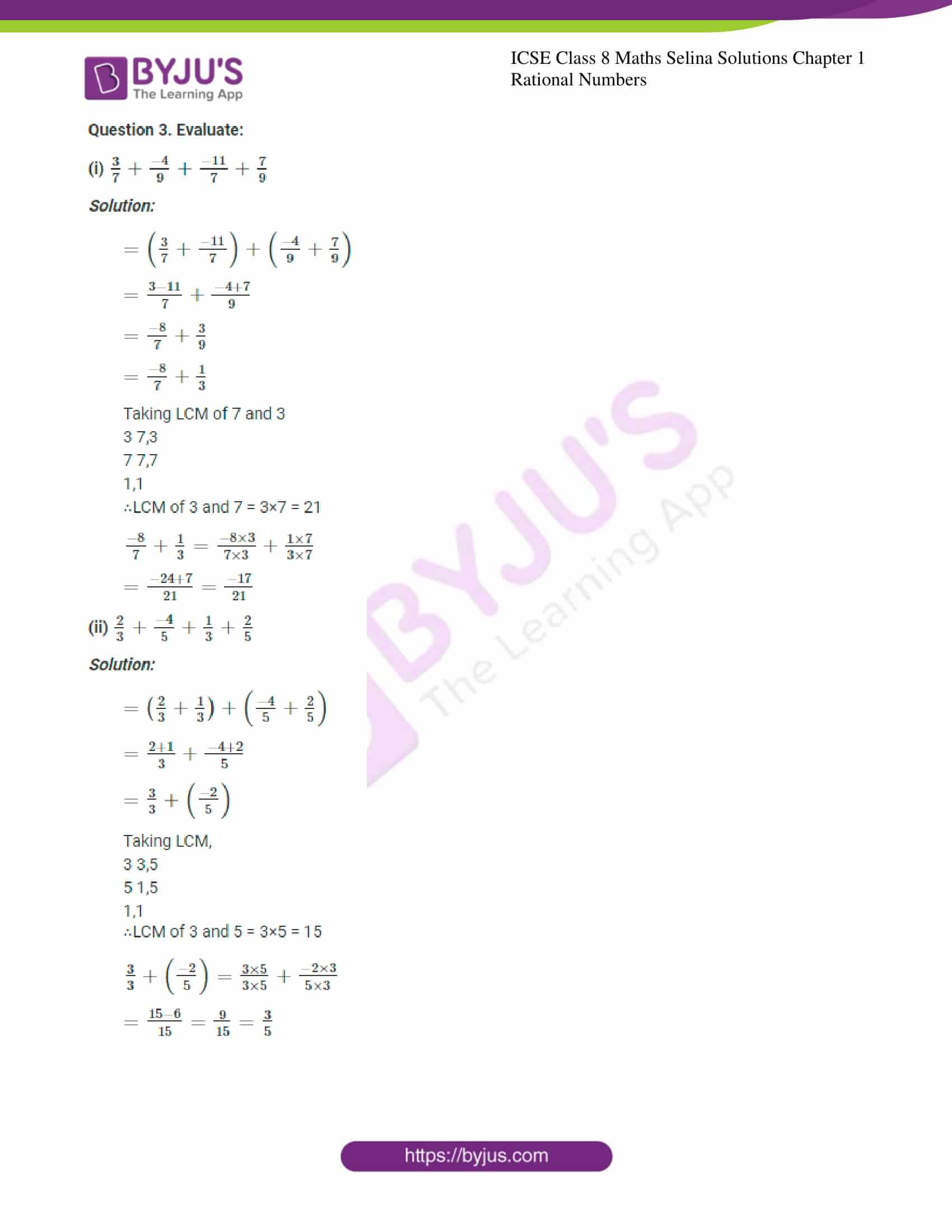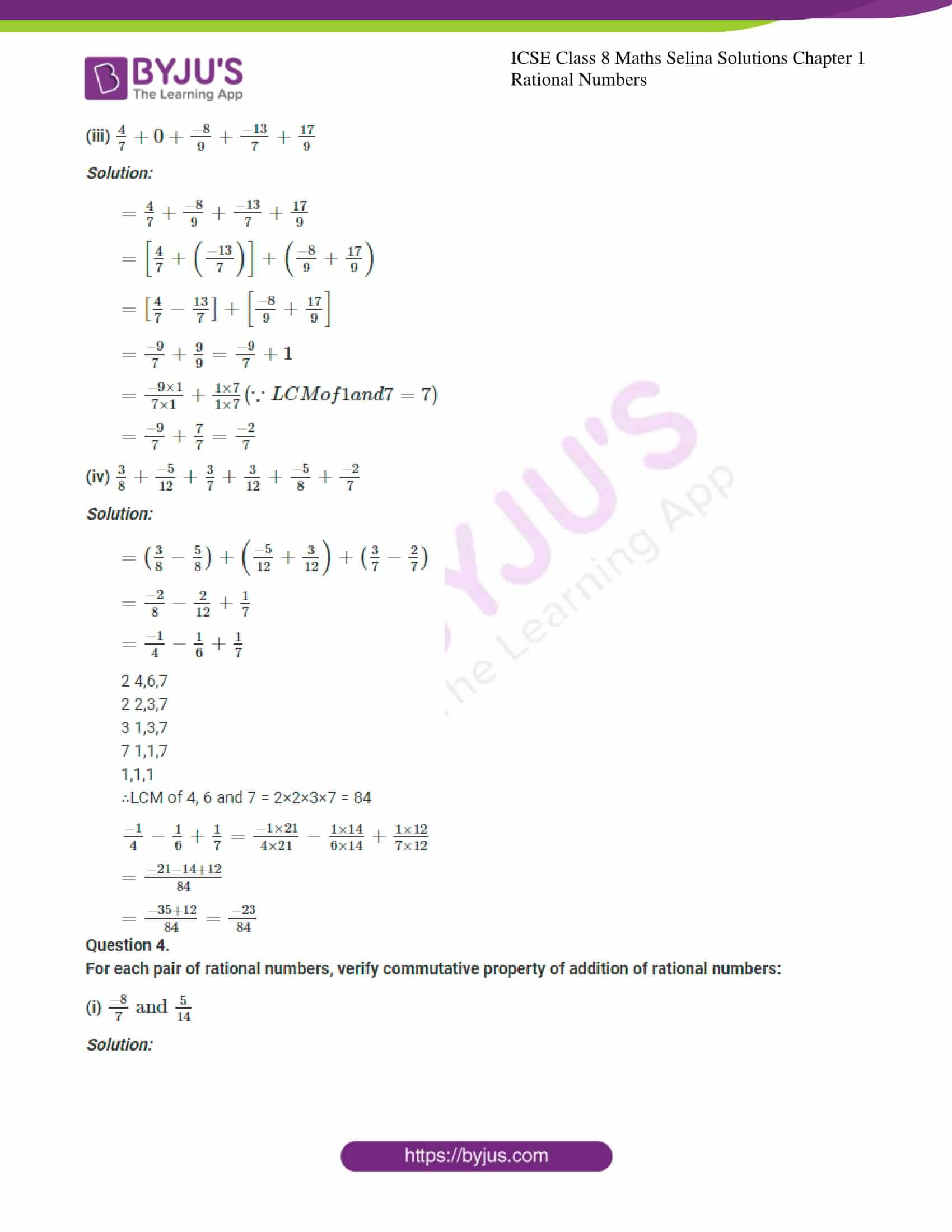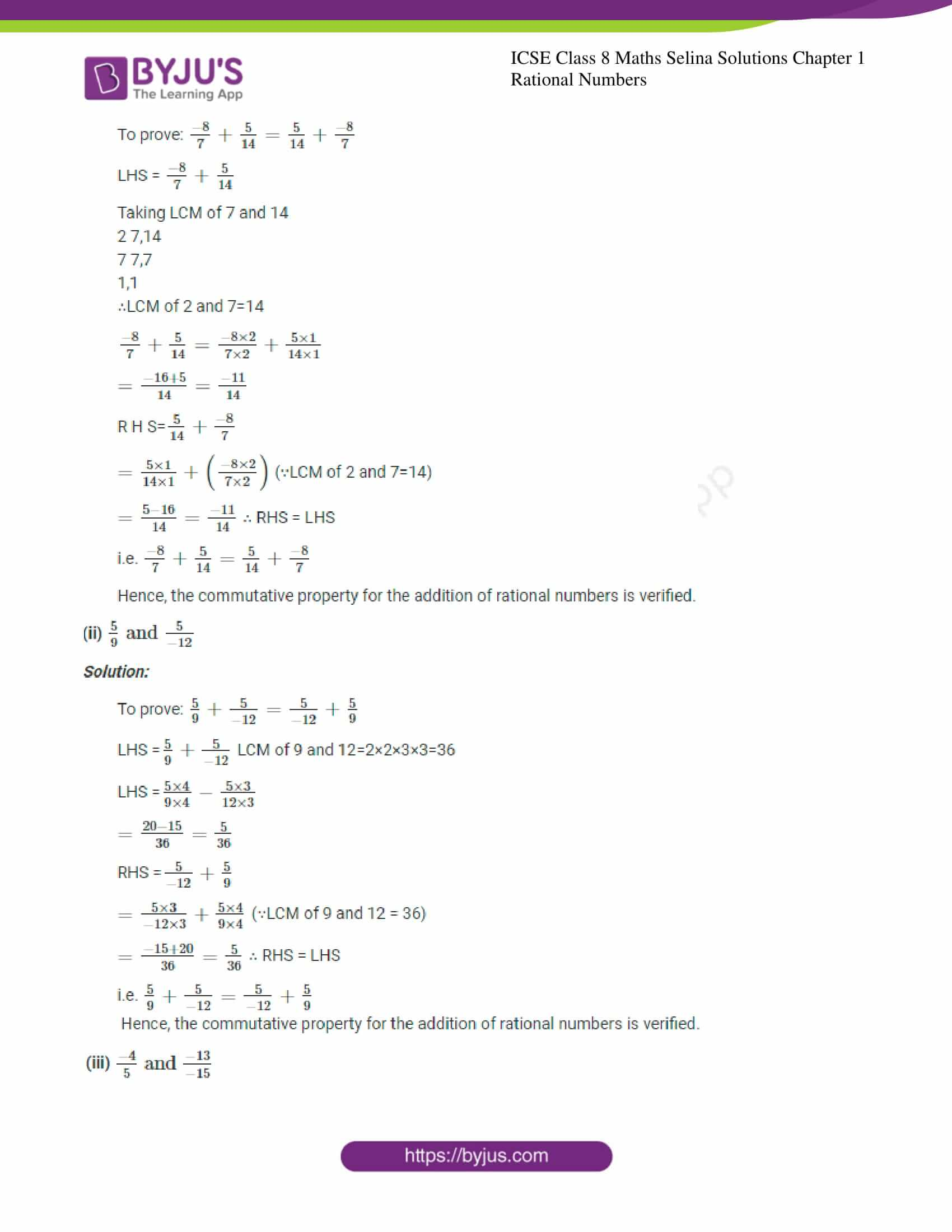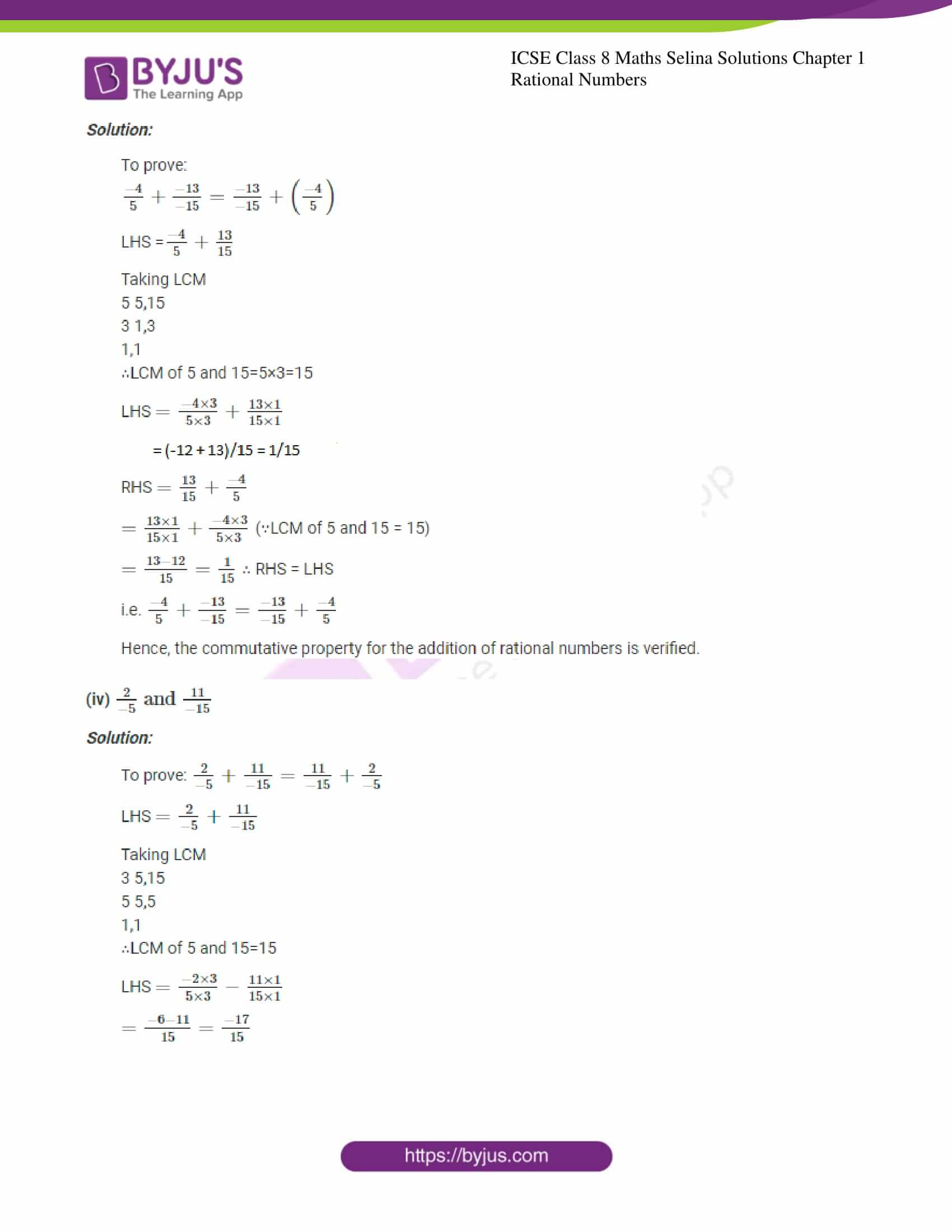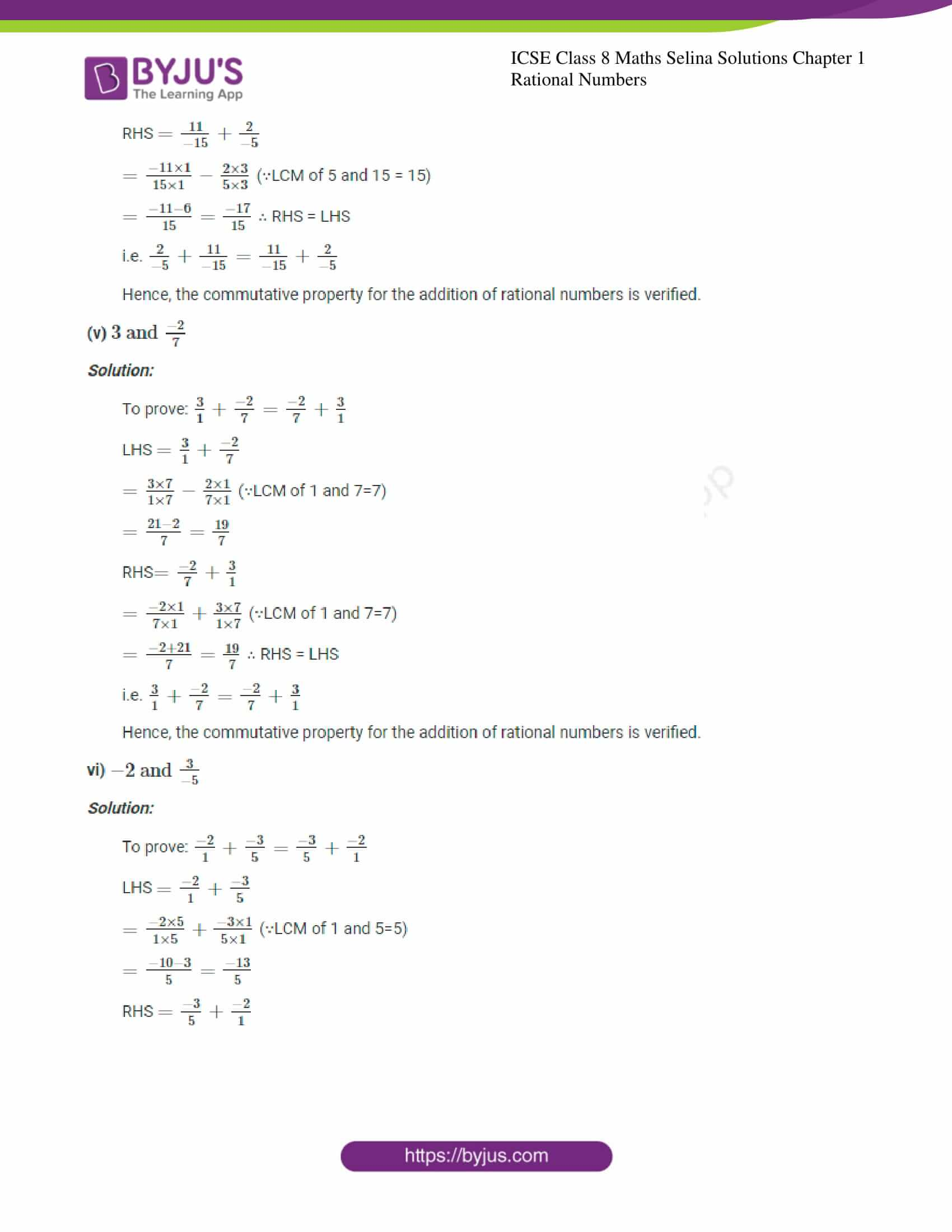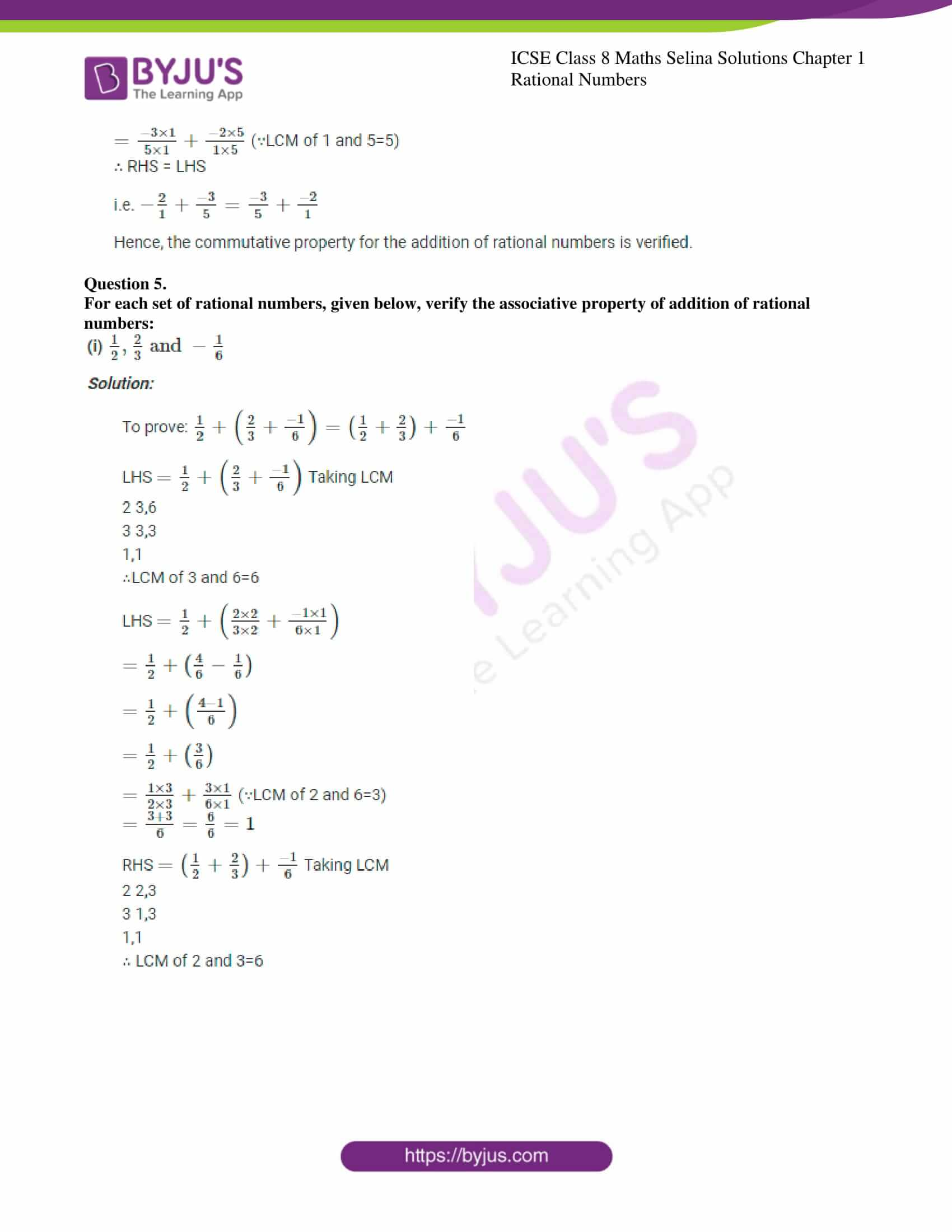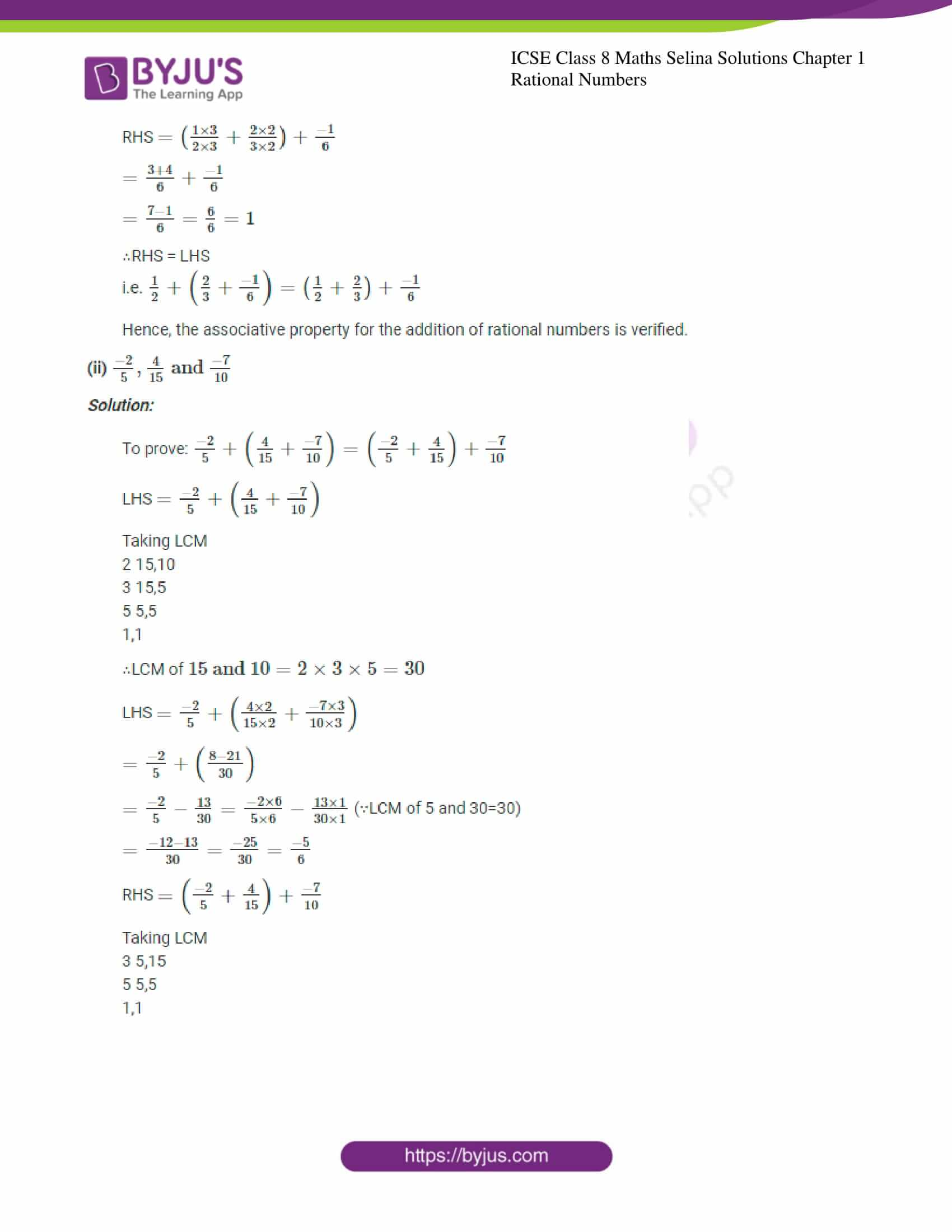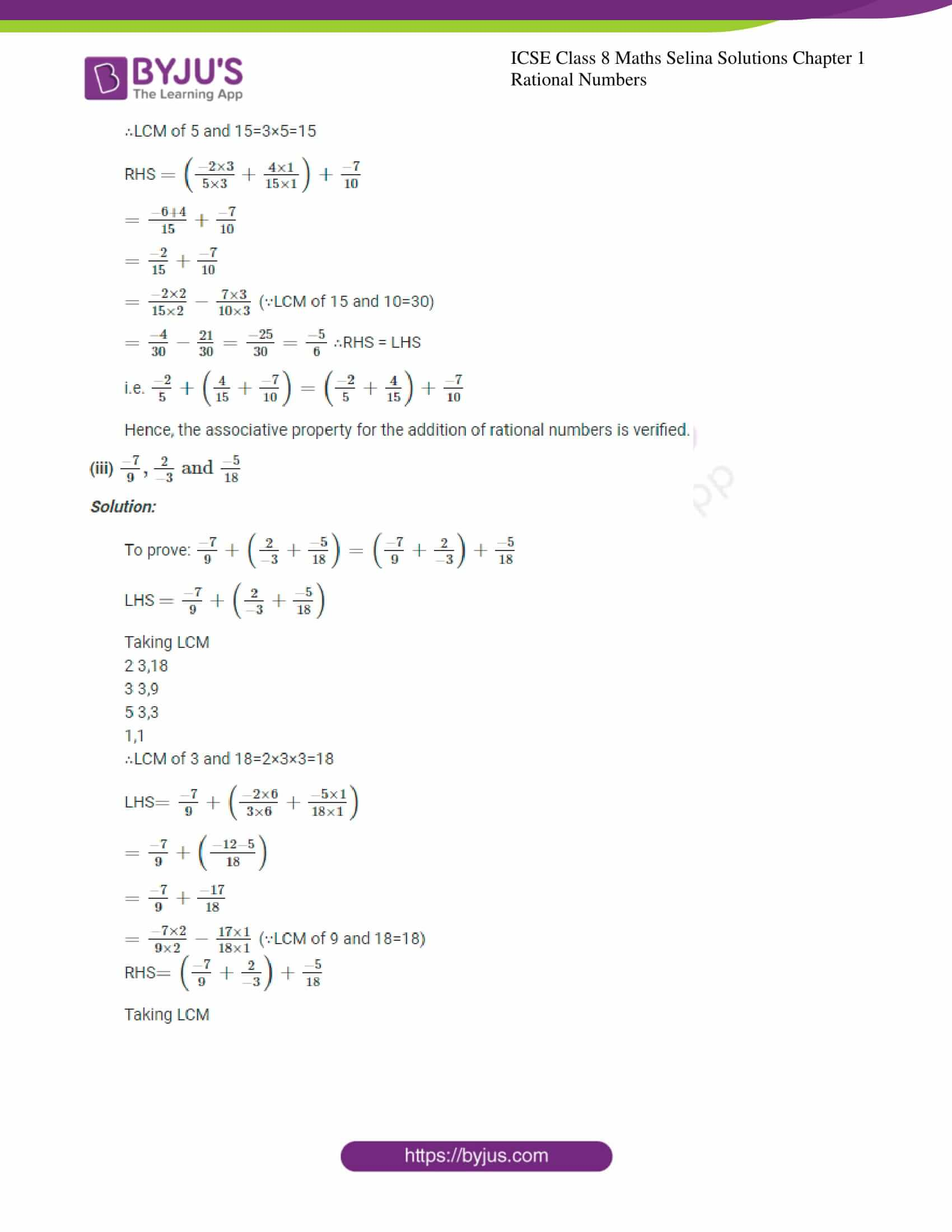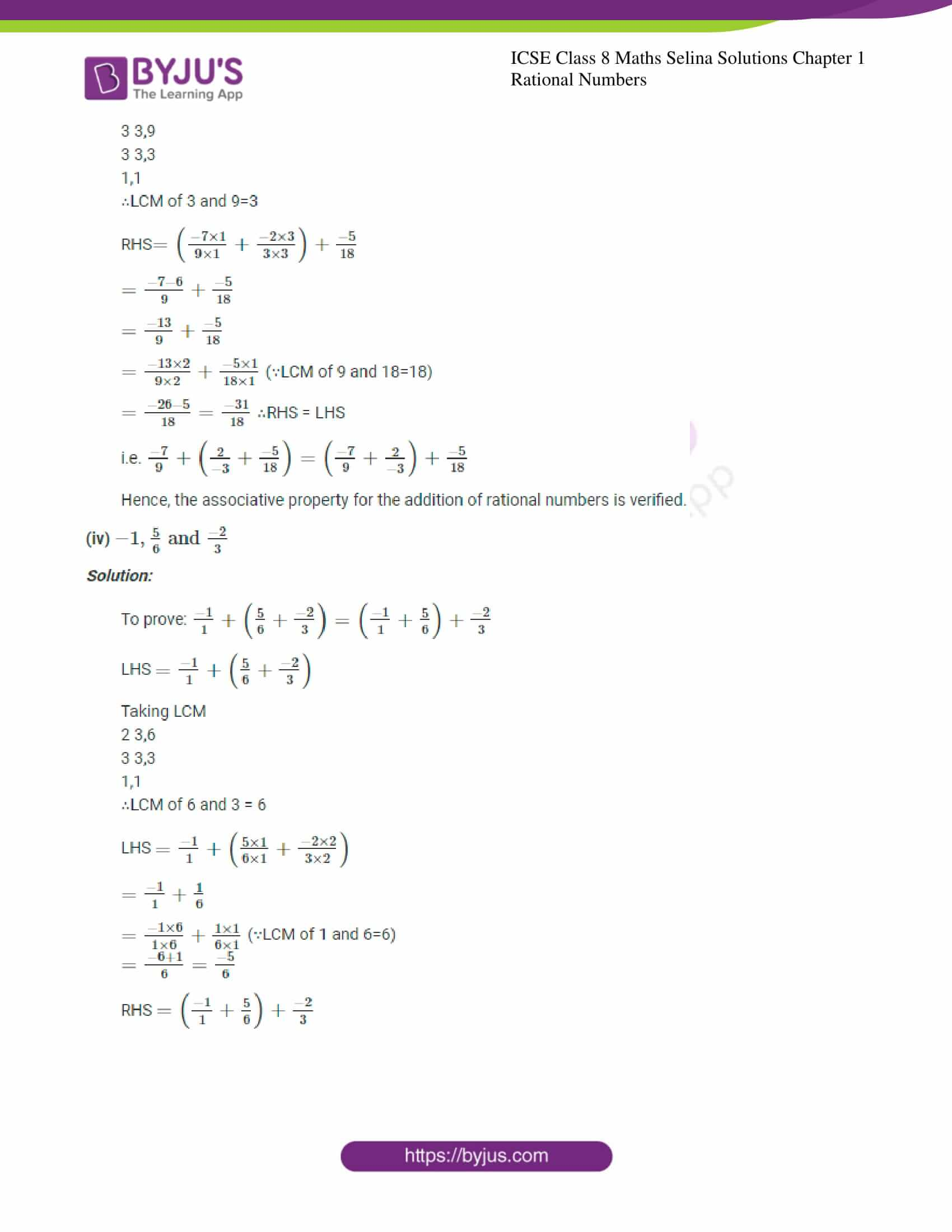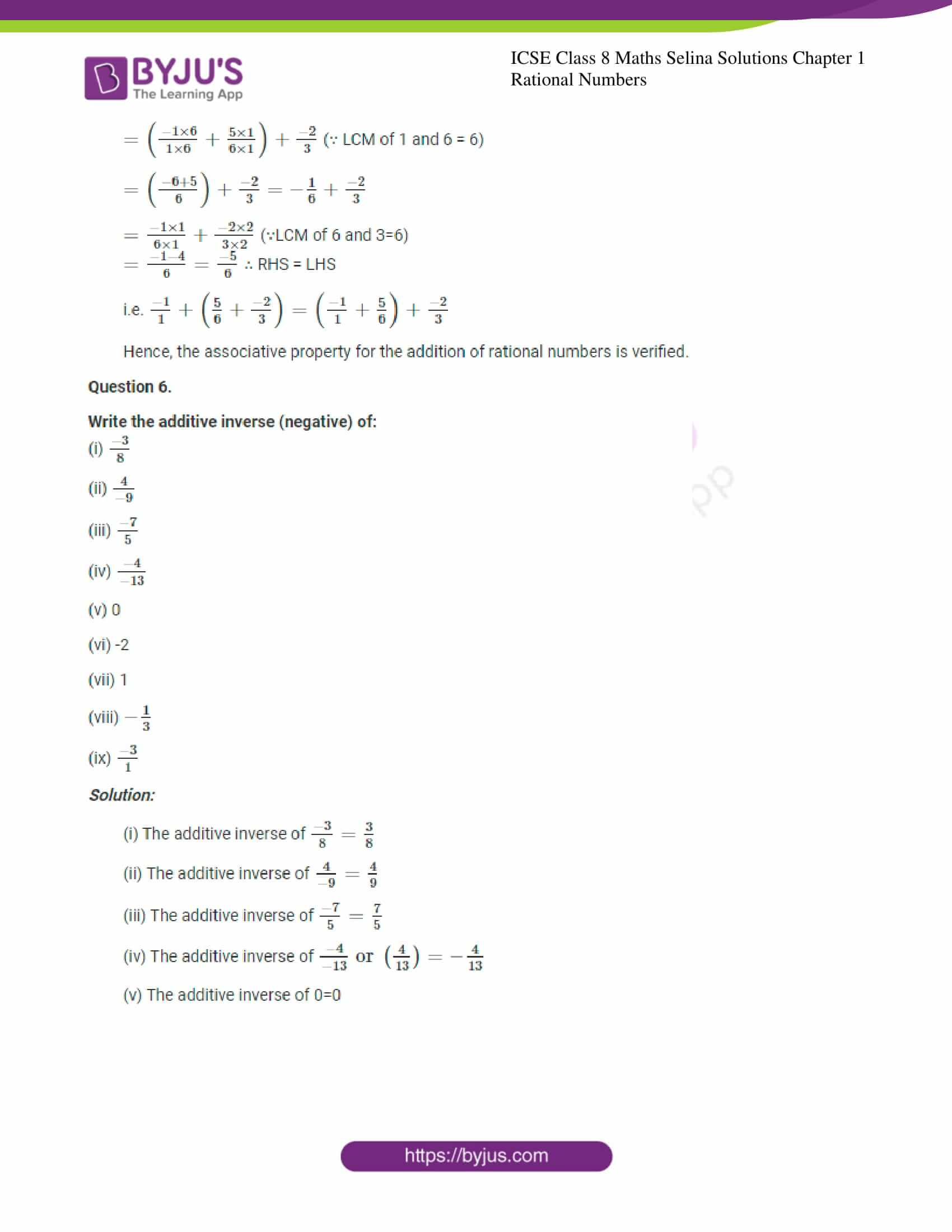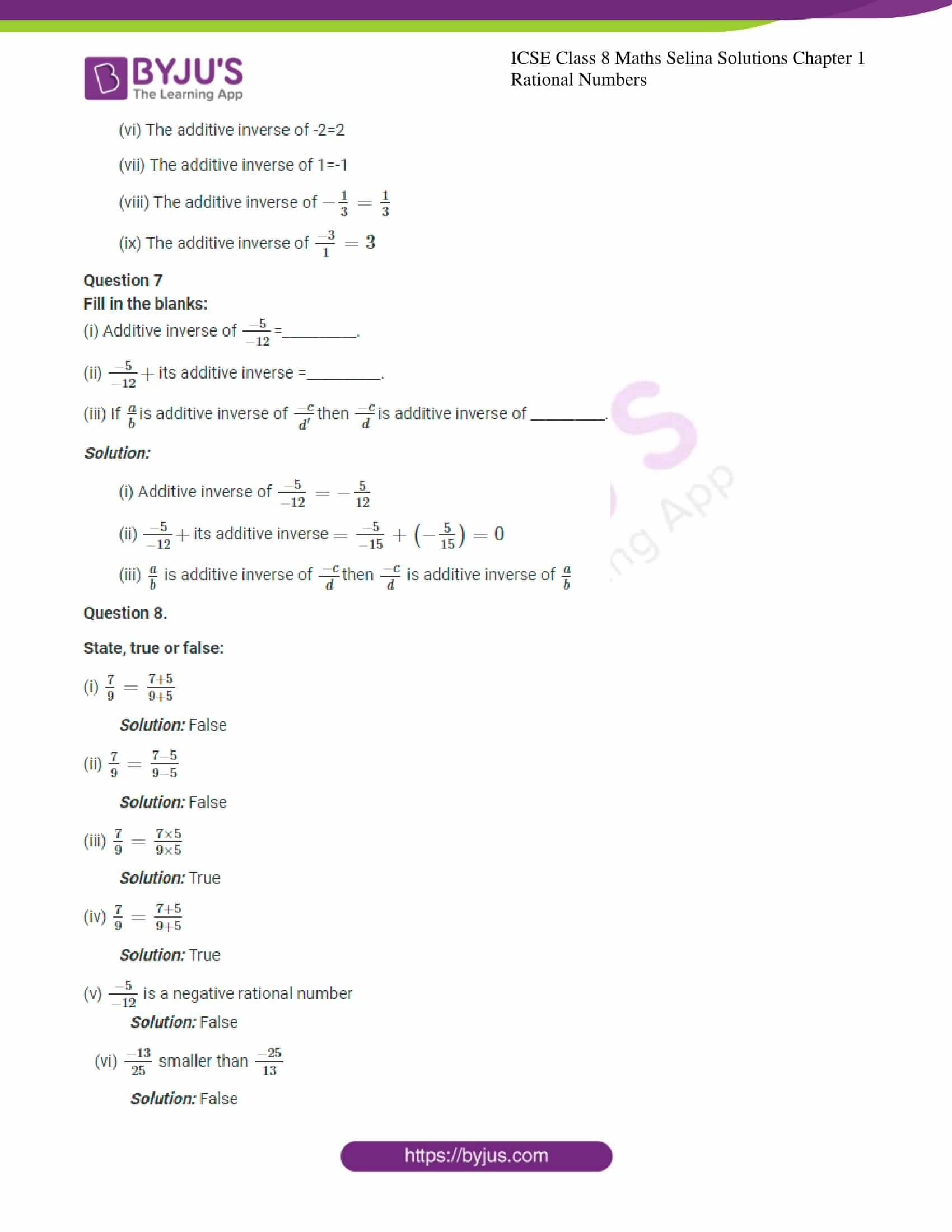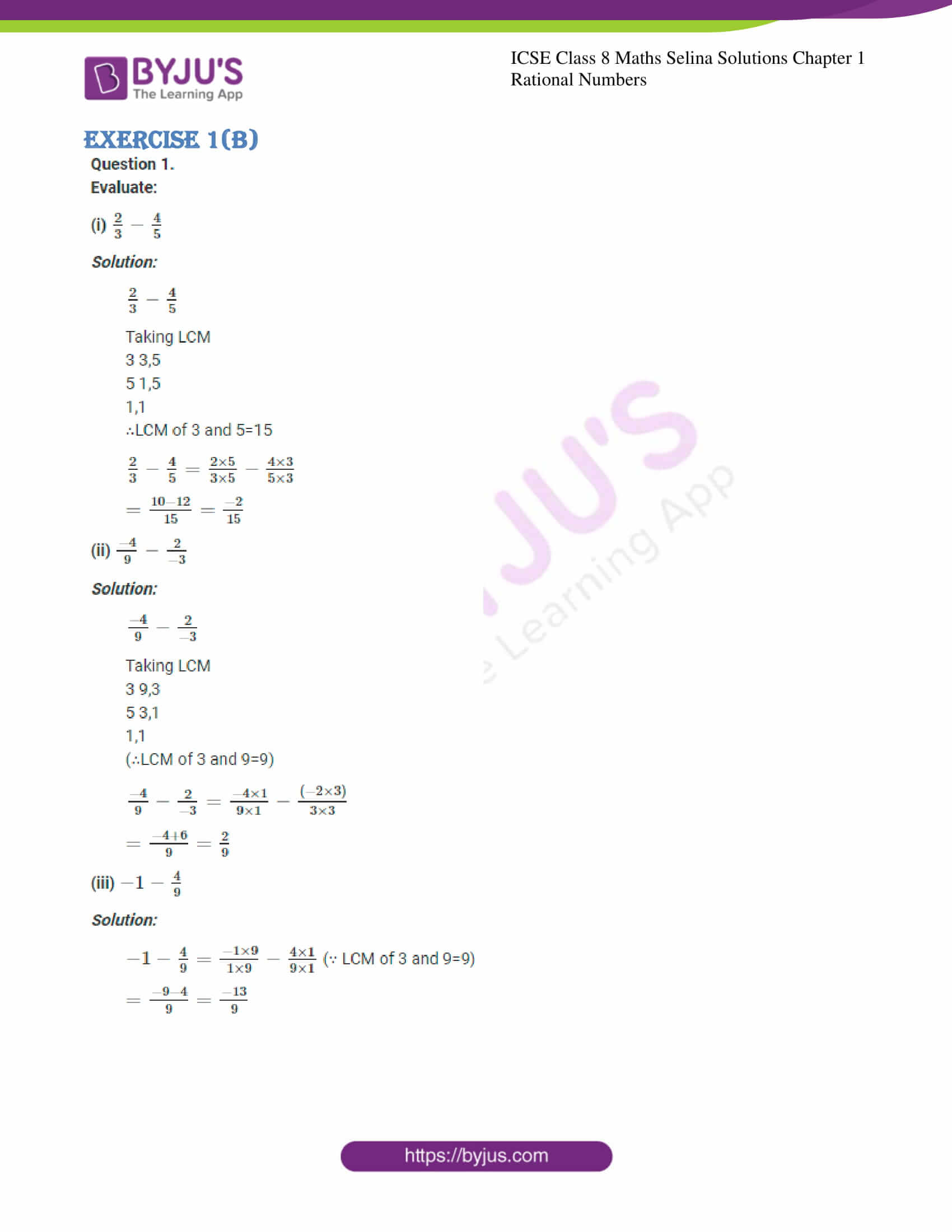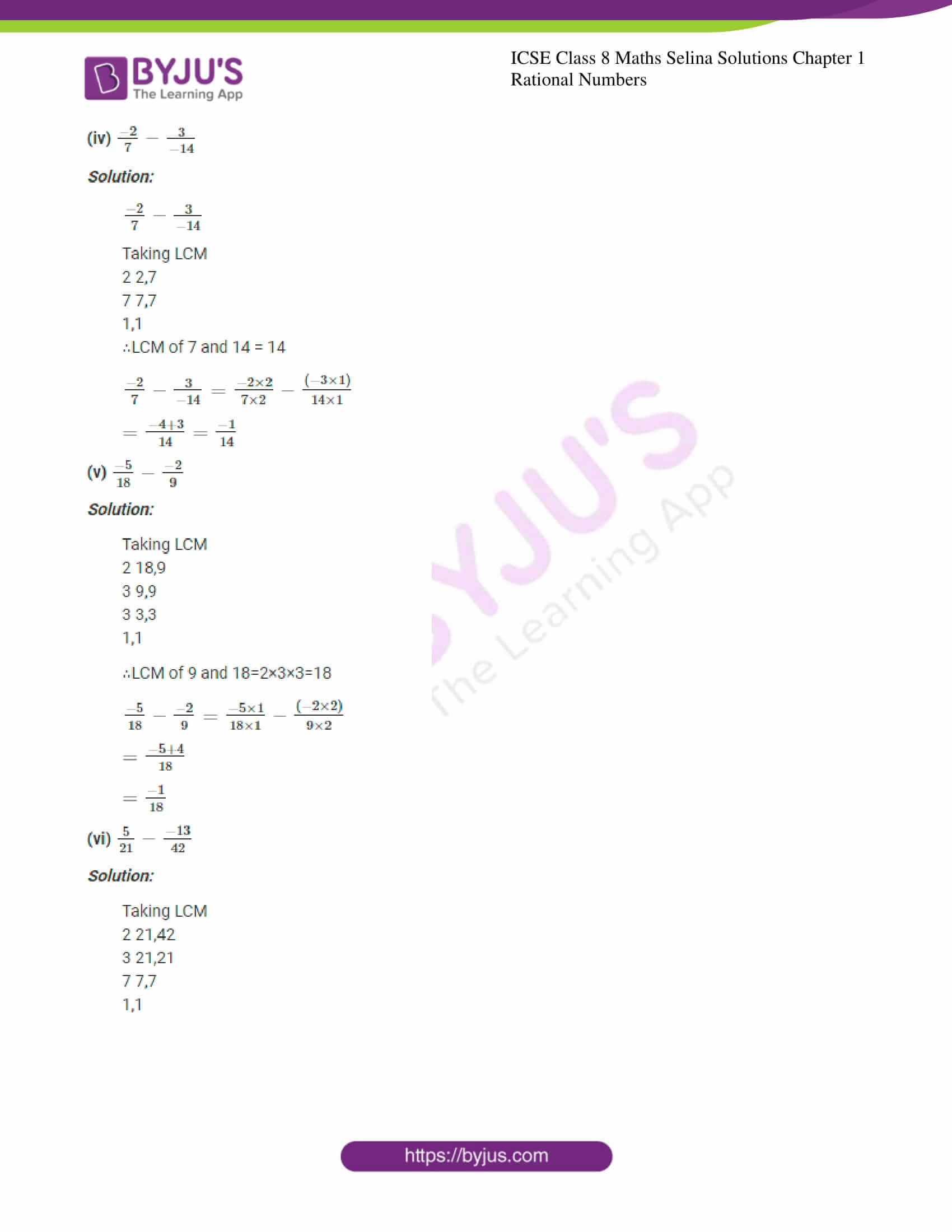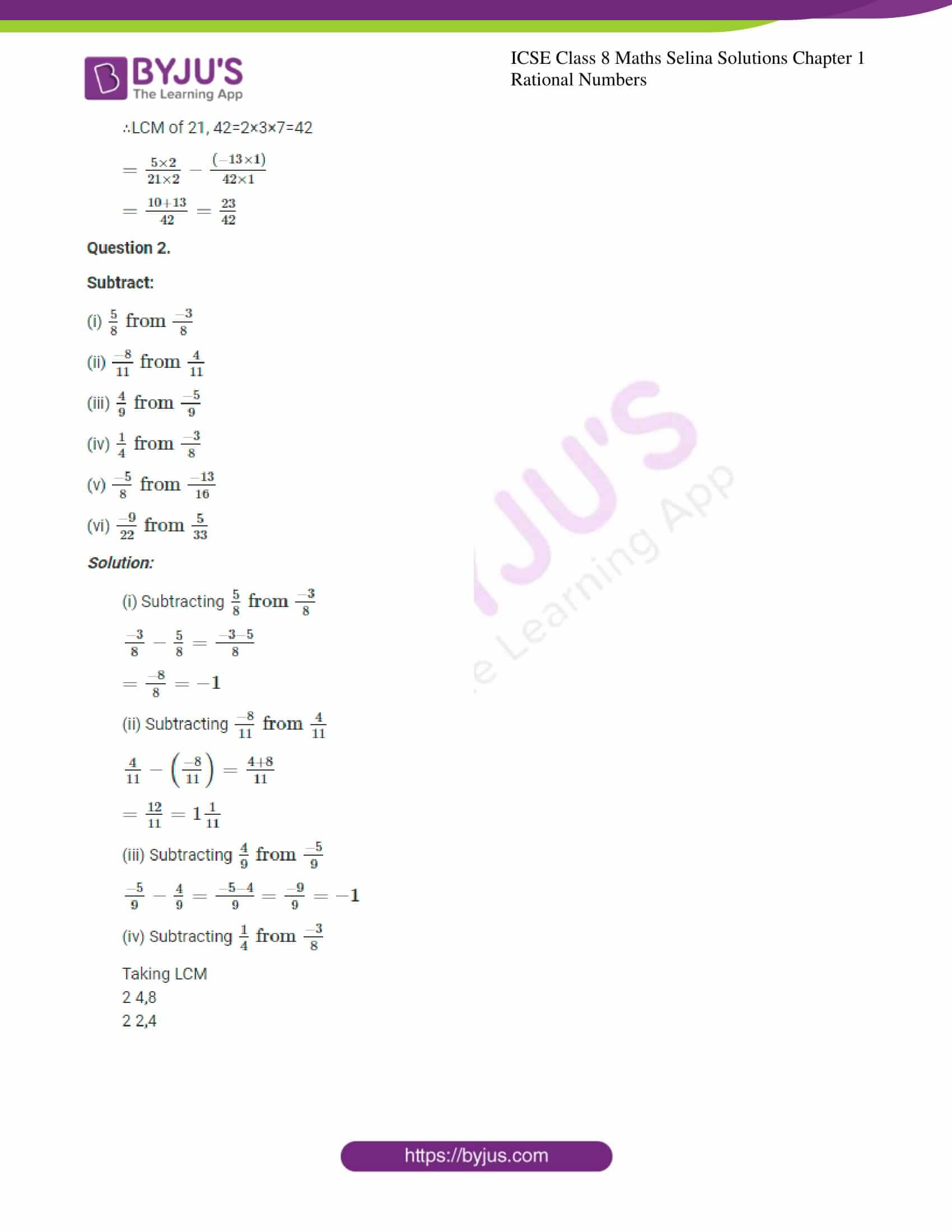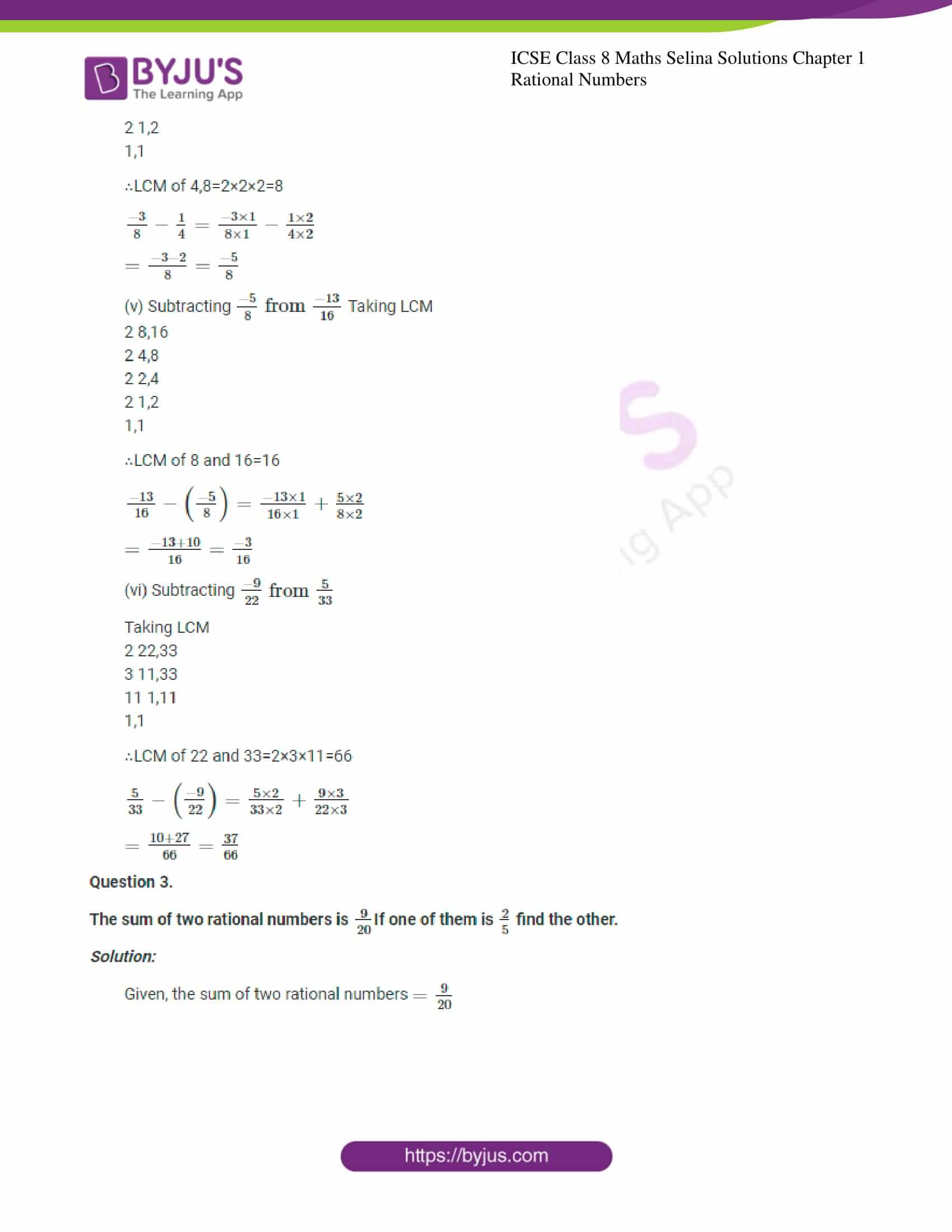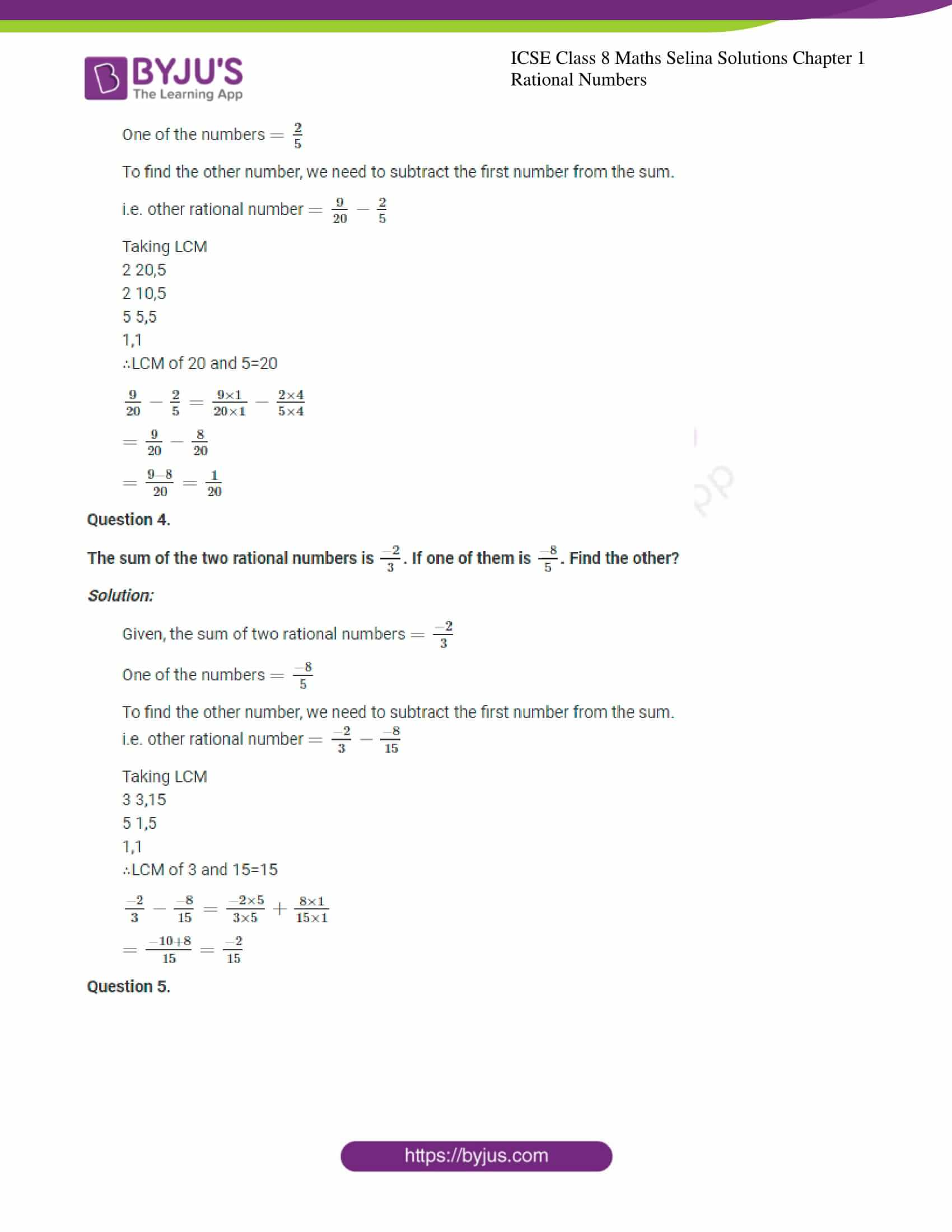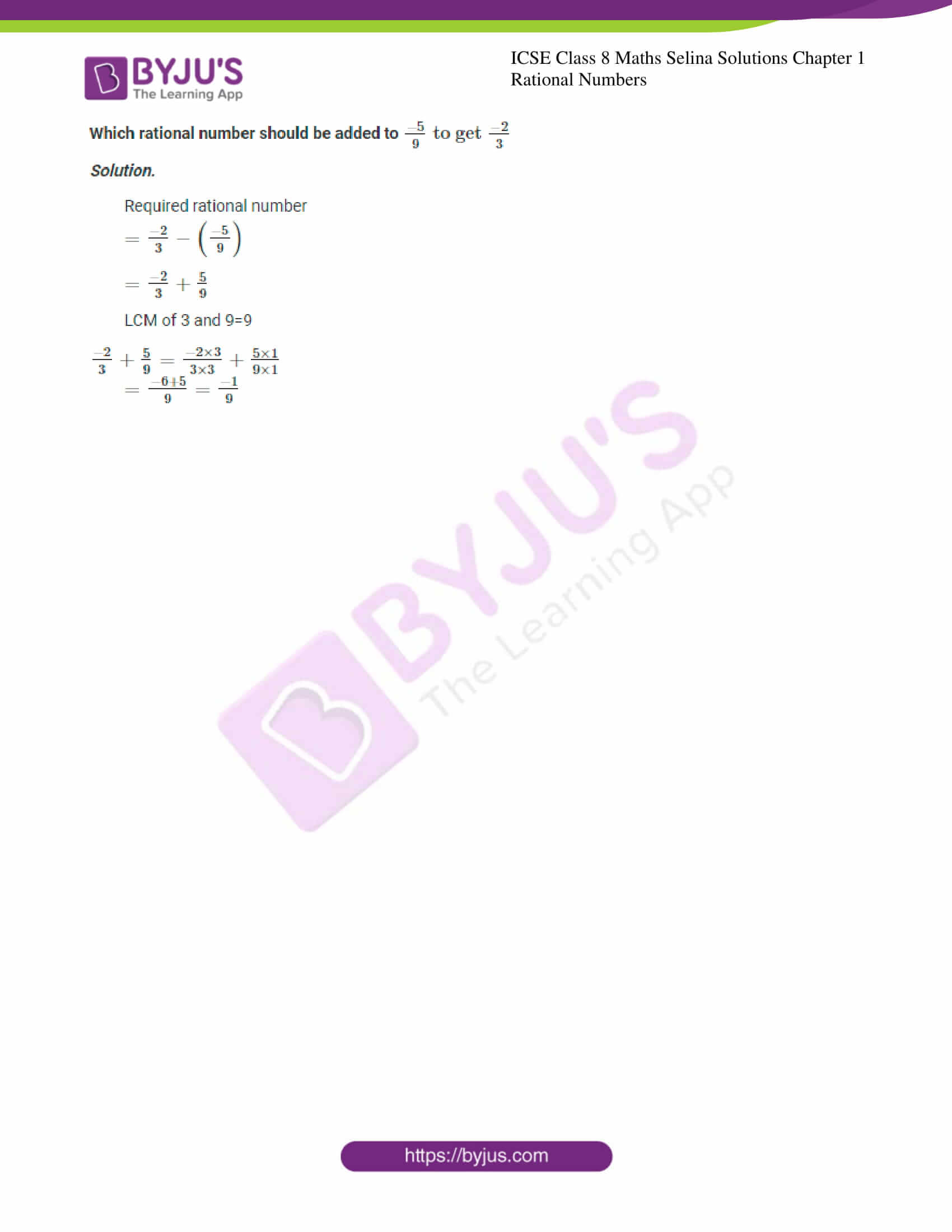### ICSE Class 8 Maths Selina Solutions Chapter 1 Rational Numbers – Exercise 1 (A)

Question 1.

Add, each pair of rational numbers, given below, and show that their addition (sum) is also a rational number.

(i) $$\frac{-5}{8} \text { and } \frac{3}{8}$$

Solution:

$$\frac{-5}{8} \text { and } \frac{3}{8}$$

$$=\frac{-5}{8}+\frac{3}{8}$$ (∵ Denominators are same, LCM=8)

$$=\frac{-5}{8}+\frac{3}{8}=\frac{-5+3}{8}$$

$$=\frac{-2}{8}=\frac{-1}{4}$$ (Cancelling numerator and denominator by 2)

Which is a rational number.

(ii) $$\frac{-8}{13} \text { and } \frac{-4}{13}$$

Solution:

$$\frac{-8}{13} \text { and } \frac{-4}{13}$$

$$=\frac{-8}{13}+\left(\frac{-4}{13}\right)$$ (∵ Denominators are same, LCM=13)

$$\frac{-8}{13}+\left(\frac{-4}{13}\right)=\frac{-8-4}{13}=\frac{-12}{13}$$

Which is a rational number.

(iii) $$\frac{6}{11} \text { and } \frac{-9}{11}$$

Solution:

$$\frac{6}{11} \text { and } \frac{-9}{11}$$

$$=\frac{6}{11}+\left(\frac{-9}{11}\right) =\frac{6}{11}+\left(\frac{-9}{11}\right)$$ (∵ Denominators are same, ∴LCM=11)

$$=\frac{6-9}{11}=\frac{-3}{11}$$

Which is a rational number.

(iv) $$\frac{5}{-26} \text { and } \frac{8}{39}$$

Solution:

$$\frac{5}{-26} \text { and } \frac{8}{39}$$

$$=\frac{5}{-26}+\frac{8}{39}$$

Taking L.C.M.

∴LCM of 26 and 39 = 2 × 3 × 13 = 78

$$\frac{5}{-26}+\frac{8}{39}=\frac{-5 \times 3}{26 \times 3}+\frac{8 \times 2}{39 \times 2}$$

$$=\frac{-15+16}{78}$$

$$=\frac{1}{78}$$

Which is a rational number.

(v) $$\frac{5}{-6} \text { and } \frac{2}{3}$$

Solution:

$$\frac{5}{-6} \text { and } \frac{2}{3}$$

$$=\frac{-5}{6}+\frac{2}{3}$$

Taking L.C.M.
2 6,3
3 3,3
1,1
∴ LCM of 6, 3=2×3=6

$$\frac{-5}{6}+\frac{2}{3}=\frac{-5 \times 1}{6 \times 1}+\frac{2 \times 2}{3 \times 2}$$ (∵LCM of 6 and 3=6)

$$=\frac{-5+4}{6}=\frac{-1}{6}$$

Which is a rational number.

(vi) $$-2 \text { and } \frac{2}{5}$$

Solution:

$$-2 \text { and } \frac{2}{5}$$

$$=\frac{-2}{1}+\frac{2}{5} (∵LCM of 1 and 5=5)$$

$$=\frac{-2 \times 5}{1 \times 5}+\frac{2 \times 1}{5 \times 1}$$

$$=\frac{-10+2}{5}=\frac{-8}{5}$$

Which is a rational number.

(vii) $$\frac{9}{-4} \text { and } \frac{-3}{8}$$

Solution:

$$\frac{9}{-4} \text { and } \frac{-3}{8}$$

$$=\frac{-9}{4}+\left(\frac{-3}{8}\right)$$

Taking L.C.M.
2 4,8
2 2,4
2 2,2
1,1
∴LCM of 4 and 8=2×2×2=8

$$\frac{-9}{4}+\left(\frac{-3}{8}\right)=\frac{-9 \times 2}{4 \times 2}-\frac{3 \times 1}{8 \times 1}$$

$$=\frac{-18-3}{8}=\frac{-21}{8}$$

Which is a rational number.

(viii) $$\frac{7}{-18} \text { and } \frac{8}{27}$$

Solution:

$$\frac{7}{-18} \text { and } \frac{8}{27}$$

$$\frac{7}{-18}+\frac{8}{27}$$

2 18,27
3 9,27
3 3,9
3 1,3
1,1
∴LCM of 18 and 27=2×3×3×3=54

$$=\frac{-21+16}{54}=\frac{-5}{54}$$ Which is a rational number.

Question 2. Evaluate:

(i) $$\frac{5}{9}+\frac{-7}{6}$$

Solution:

$$\frac{5}{9}+\frac{-7}{6}$$ Taking L.C.M.
2 9,6
3 9,3
3 3,1
1,1
∴LCM of 9 and 6=2×3×3=18

$$\frac{5}{9}+\frac{-7}{6}$$

$$=\frac{5 \times 2}{9 \times 2}-\frac{7 \times 3}{6 \times 3}$$

(∵LCM of 9 and 6=18)

$$=\frac{10-21}{18}=\frac{-11}{8}$$

(ii) $$4+\frac{3}{-5}$$

Solution:

$$4+\frac{3}{-5}$$

$$4+\frac{3}{-5}=\frac{4}{1}+\left(\frac{-3}{5}\right)$$

$$=\frac{4}{1}-\frac{3}{5}$$

Taking L.C.M.
LCM of 1 and 5=5
$$4+\frac{3}{-5}=\frac{4 \times 5}{1 \times 5}-\frac{3 \times 1}{5 \times 1}$$

$$=\frac{20-3}{5}=\frac{17}{5}=3 \frac{2}{5}$$ (Displaying the answer in mixed fraction)

(iii) $$\frac{1}{-15}+\frac{5}{-12}$$

Solution:

$$\frac{1}{-15}+\frac{5}{-12}$$

$$=\frac{-1}{15}+\left(\frac{-5}{12}\right)$$

$$=\frac{-1}{15}-\frac{5}{12}$$

Taking L.C.M.
2 15,2
2 15,6
3 15,3
5 5,1
1,1

∴LCM of 15 and 12=2×2×3×5=60
$$=\frac{-1 \times 4}{15 \times 4}-\frac{5 \times 5}{12 \times 5}$$

LCM of 15 and 12 = 60

$$\frac{1}{-15}+\frac{5}{-12}=\frac{-4-25}{60}=\frac{-29}{60}$$

(iv) $$\frac{5}{9}+\frac{3}{-4}$$

Solution:

$$\frac{5}{9}+\frac{3}{-4}$$

$$=\frac{5}{9}-\frac{3}{4}$$ (Manipulating the signs)

LCM of 9 and 4=2×2×3×3=36

$$\frac{5}{9}+\frac{3}{-4}=\frac{5 \times 4}{9 \times 4}-\frac{3 \times 9}{4 \times 9}$$

$$=\frac{20-27}{36}=\frac{-7}{36}$$

$$=\frac{-7}{36}$$

(v) $$\frac{-8}{9}+\frac{-5}{12}$$

Solution:

$$\frac{-8}{9}+\frac{-5}{12}$$ Taking L.C.M.
2 9,12
2 9,6
3 9,3
3 3,1
1,1

∴ LCM of 9, 12=2×2×3×3=36
$$\frac{-8}{9}+\frac{-5}{12}=\frac{-8 \times 4}{9 \times 4}-\frac{5 \times 3}{12 \times 3}$$

$$=\frac{-32-15}{36}$$

$$=\frac{-47}{36}$$

(vi) $$0+\frac{-2}{7}$$

Solution:

$$0+\frac{-2}{7}$$ LCM of 0 and 7=7
By cross multiplying
$$0+\frac{-2}{7}=\frac{0 \times 7}{1 \times 7}-\frac{2 \times 1}{7 \times 1}$$

$$=\frac{0-2}{7}=\frac{-2}{7}$$

(vii) $$\frac{5}{-11}+0$$

Solution:

$$\frac{5}{-11}+0$$

LCM of 0 and 11=11
By cross multiplying
$$\frac{5}{-11}+0=\frac{-5 \times 1}{11 \times 1}+\frac{0 \times 11}{1 \times 11}$$

$$=\frac{-5+0}{11}=\frac{-5}{11}$$

(viii) $$2+\frac{-3}{5}$$

Solution:

$$=\frac{2}{1}-\frac{3}{5}$$ LCM of 1 and 5=5
$$=\frac{2 \times 5}{1 \times 5}-\frac{3 \times 1}{5 \times 1}$$

$$=\frac{10-3}{5}=\frac{7}{5}=1 \frac{2}{5}$$

(ix) $$\frac{4}{-9}+1$$

Solution:

$$\frac{4}{-9}+1$$

LCM of 9 and 1=9
$$\frac{-4}{9}+\frac{1}{1}=\frac{-4 \times 1}{9 \times 1}+\frac{1 \times 9}{1 \times 9}$$

$$=\frac{-4+9}{9}=\frac{5}{9}$$

Question 3. Evaluate:

(i) $$\frac{3}{7}+\frac{-4}{9}+\frac{-11}{7}+\frac{7}{9}$$

Solution:

$$=\left(\frac{3}{7}+\frac{-11}{7}\right)+\left(\frac{-4}{9}+\frac{7}{9}\right)$$

$$=\frac{3-11}{7}+\frac{-4+7}{9}$$

$$=\frac{-8}{7}+\frac{3}{9}$$

$$=\frac{-8}{7}+\frac{1}{3}$$

Taking LCM of 7 and 3
3 7,3
7 7,7
1,1
∴LCM of 3 and 7 = 3×7 = 21

$$\frac{-8}{7}+\frac{1}{3}=\frac{-8 \times 3}{7 \times 3}+\frac{1 \times 7}{3 \times 7}$$

$$=\frac{-24+7}{21}=\frac{-17}{21}$$

(ii) $$\frac{2}{3}+\frac{-4}{5}+\frac{1}{3}+\frac{2}{5}$$

Solution:

$$=\left(\frac{2}{3}+\frac{1}{3}\right)+\left(\frac{-4}{5}+\frac{2}{5}\right)$$

$$=\frac{2+1}{3}+\frac{-4+2}{5}$$

$$=\frac{3}{3}+\left(\frac{-2}{5}\right)$$

Taking LCM,
3 3,5
5 1,5
1,1
∴LCM of 3 and 5 = 3×5 = 15

$$\frac{3}{3}+\left(\frac{-2}{5}\right)=\frac{3 \times 5}{3 \times 5}+\frac{-2 \times 3}{5 \times 3}$$

$$=\frac{15-6}{15}=\frac{9}{15}=\frac{3}{5}$$

(iii) $$\frac{4}{7}+0+\frac{-8}{9}+\frac{-13}{7}+\frac{17}{9}$$

Solution:

$$=\frac{4}{7}+\frac{-8}{9}+\frac{-13}{7}+\frac{17}{9}$$

$$=\left[\frac{4}{7}+\left(\frac{-13}{7}\right)\right]+\left(\frac{-8}{9}+\frac{17}{9}\right)$$

$$=\left[\frac{4}{7}-\frac{13}{7}\right]+\left[\frac{-8}{9}+\frac{17}{9}\right]$$

$$=\frac{-9}{7}+\frac{9}{9}=\frac{-9}{7}+1$$

$$=\frac{-9 \times 1}{7 \times 1}+\frac{1 \times 7}{1 \times 7} (∵LCM of 1 and 7=7)$$

$$=\frac{-9}{7}+\frac{7}{7}=\frac{-2}{7}$$

(iv) $$\frac{3}{8}+\frac{-5}{12}+\frac{3}{7}+\frac{3}{12}+\frac{-5}{8}+\frac{-2}{7}$$

Solution:

$$=\left(\frac{3}{8}-\frac{5}{8}\right)+\left(\frac{-5}{12}+\frac{3}{12}\right)+\left(\frac{3}{7}-\frac{2}{7}\right)$$

$$=\frac{-2}{8}-\frac{2}{12}+\frac{1}{7}$$

$$=\frac{-1}{4}-\frac{1}{6}+\frac{1}{7}$$

2 4,6,7
2 2,3,7
3 1,3,7
7 1,1,7
1,1,1
∴LCM of 4, 6 and 7 = 2×2×3×7 = 84

$$\frac{-1}{4}-\frac{1}{6}+\frac{1}{7}=\frac{-1 \times 21}{4 \times 21}-\frac{1 \times 14}{6 \times 14}+\frac{1 \times 12}{7 \times 12}$$

$$=\frac{-21-14+12}{84}$$

$$=\frac{-35+12}{84}=\frac{-23}{84}$$

Question 4.
For each pair of rational numbers, verify commutative property of addition of rational numbers:

(i) $$\frac{-8}{7} \text { and } \frac{5}{14}$$

Solution:

To prove: $$\frac{-8}{7}+\frac{5}{14}=\frac{5}{14}+\frac{-8}{7}$$

LHS = $$\frac{-8}{7}+\frac{5}{14}$$

Taking LCM of 7 and 14
2 7,14
7 7,7
1,1
∴LCM of 2 and 7=14

$$\frac{-8}{7}+\frac{5}{14}=\frac{-8 \times 2}{7 \times 2}+\frac{5 \times 1}{14 \times 1}$$

$$=\frac{-16+5}{14}=\frac{-11}{14}$$

R H S=$$\frac{5}{14}+\frac{-8}{7}$$

$$=\frac{5 \times 1}{14 \times 1}+\left(\frac{-8 \times 2}{7 \times 2}\right)$$ (∵LCM of 2 and 7=14)

$$=\frac{5-16}{14}=\frac{-11}{14}$$ ∴ RHS = LHS

i.e. $$\frac{-8}{7}+\frac{5}{14}=\frac{5}{14}+\frac{-8}{7}$$

Hence, the commutative property for the addition of rational numbers is verified.

(ii) $$\frac{5}{9} \text { and } \frac{5}{-12}$$

Solution:

To prove: $$\frac{5}{9}+\frac{5}{-12}=\frac{5}{-12}+\frac{5}{9}$$

LHS =$$\frac{5}{9}+\frac{5}{-12}$$ LCM of 9 and 12=2×2×3×3=36

LHS =$$\frac{5 \times 4}{9 \times 4}-\frac{5 \times 3}{12 \times 3}$$

$$=\frac{20-15}{36}=\frac{5}{36}$$

RHS =$$\frac{5}{-12}+\frac{5}{9}$$

$$=\frac{5 \times 3}{-12 \times 3}+\frac{5 \times 4}{9 \times 4}$$ (∵LCM of 9 and 12 = 36)

$$=\frac{-15+20}{36}=\frac{5}{36}$$ ∴ RHS = LHS

i.e. $$\frac{5}{9}+\frac{5}{-12}=\frac{5}{-12}+\frac{5}{9}$$

Hence, the commutative property for the addition of rational numbers is verified.

(iii) $$\frac{-4}{5} \text { and } \frac{-13}{-15}$$

Solution:

To prove:
$$\frac{-4}{5}+\frac{-13}{-15}=\frac{-13}{-15}+\left(\frac{-4}{5}\right)$$

LHS =$$\frac{-4}{5}+\frac{13}{15}$$

Taking LCM
5 5,15
3 1,3
1,1
∴LCM of 5 and 15=5×3=15

LHS $$=\frac{-4 \times 3}{5 \times 3}+\frac{13 \times 1}{15 \times 1}$$

$$=\frac{-12+13}{15}=\frac{1}{15}$$

RHS $$=\frac{13}{15}+\frac{-4}{5}$$

$$=\frac{13 \times 1}{15 \times 1}+\frac{-4 \times 3}{5 \times 3}$$ (∵LCM of 5 and 15 = 15)

$$=\frac{13-12}{15}=\frac{1}{15}$$ ∴ RHS = LHS

i.e. $$\frac{-4}{5}+\frac{-13}{-15}=\frac{-13}{-15}+\frac{-4}{5}$$

Hence, the commutative property for the addition of rational numbers is verified.

(iv) $$\frac{2}{-5} \text { and } \frac{11}{-15}$$

Solution:

To prove: $$\frac{2}{-5}+\frac{11}{-15}=\frac{11}{-15}+\frac{2}{-5}$$

LHS $$=\frac{2}{-5}+\frac{11}{-15}$$

Taking LCM
3 5,15
5 5,5
1,1
∴LCM of 5 and 15=15

LHS $$=\frac{-2 \times 3}{5 \times 3}-\frac{11 \times 1}{15 \times 1}$$

$$=\frac{-6-11}{15}=\frac{-17}{15}$$

RHS $$=\frac{11}{-15}+\frac{2}{-5}$$

$$=\frac{-11 \times 1}{15 \times 1}-\frac{2 \times 3}{5 \times 3}$$ (∵LCM of 5 and 15 = 15)

$$=\frac{-11-6}{15}=\frac{-17}{15}$$ ∴ RHS = LHS

i.e. $$\frac{2}{-5}+\frac{11}{-15}=\frac{11}{-15}+\frac{2}{-5}$$

Hence, the commutative property for the addition of rational numbers is verified.

(v) $$3 \text { and } \frac{-2}{7}$$

Solution:

To prove: $$\frac{3}{1}+\frac{-2}{7}=\frac{-2}{7}+\frac{3}{1}$$

LHS $$=\frac{3}{1}+\frac{-2}{7}$$

$$=\frac{3 \times 7}{1 \times 7}-\frac{2 \times 1}{7 \times 1}$$ (∵LCM of 1 and 7=7)

$$=\frac{21-2}{7}=\frac{19}{7}$$

RHS$$=\frac{-2}{7}+\frac{3}{1}$$

$$=\frac{-2 \times 1}{7 \times 1}+\frac{3 \times 7}{1 \times 7}$$ (∵LCM of 1 and 7=7)

$$=\frac{-2+21}{7}=\frac{19}{7}$$ ∴ RHS = LHS

i.e. $$\frac{3}{1}+\frac{-2}{7}=\frac{-2}{7}+\frac{3}{1}$$

Hence, the commutative property for the addition of rational numbers is verified.

vi) $$-2 \text { and } \frac{3}{-5}$$

Solution:

To prove: $$\frac{-2}{1}+\frac{-3}{5}=\frac{-3}{5}+\frac{-2}{1}$$

LHS $$=\frac{-2}{1}+\frac{-3}{5}$$

$$=\frac{-2 \times 5}{1 \times 5}+\frac{-3 \times 1}{5 \times 1}$$ (∵LCM of 1 and 5=5)

$$=\frac{-10-3}{5}=\frac{-13}{5}$$

RHS $$=\frac{-3}{5}+\frac{-2}{1}$$

$$=\frac{-3 \times 1}{5 \times 1}+\frac{-2 \times 5}{1 \times 5}$$ (∵LCM of 1 and 5=5)
∴ RHS = LHS

i.e. $$-\frac{2}{1}+\frac{-3}{5}=\frac{-3}{5}+\frac{-2}{1}$$

Hence, the commutative property for the addition of rational numbers is verified.

Question 5.

For each set of rational numbers, given below, verify the associative property of addition of rational numbers:

(i) $$\frac{1}{2}, \frac{2}{3} \text { and }-\frac{1}{6}$$

Solution:

To prove: $$\frac{1}{2}+\left(\frac{2}{3}+\frac{-1}{6}\right)=\left(\frac{1}{2}+\frac{2}{3}\right)+\frac{-1}{6}$$

LHS $$=\frac{1}{2}+\left(\frac{2}{3}+\frac{-1}{6}\right)$$ Taking LCM
2 3,6
3 3,3
1,1
∴LCM of 3 and 6=6

LHS $$=\frac{1}{2}+\left(\frac{2 \times 2}{3 \times 2}+\frac{-1 \times 1}{6 \times 1}\right)$$

$$=\frac{1}{2}+\left(\frac{4}{6}-\frac{1}{6}\right)$$

$$=\frac{1}{2}+\left(\frac{4-1}{6}\right)$$

$$=\frac{1}{2}+\left(\frac{3}{6}\right)$$

$$=\frac{1 \times 3}{2 \times 3}+\frac{3 \times 1}{6 \times 1}$$ (∵LCM of 2 and 6=3)
$$=\frac{3+3}{6}=\frac{6}{6}=1$$

RHS $$=\left(\frac{1}{2}+\frac{2}{3}\right)+\frac{-1}{6}$$ Taking LCM
2 2,3
3 1,3
1,1
∴ LCM of 2 and 3=6

RHS $$=\left(\frac{1 \times 3}{2 \times 3}+\frac{2 \times 2}{3 \times 2}\right)+\frac{-1}{6}$$

$$=\frac{3+4}{6}+\frac{-1}{6}$$

$$=\frac{7-1}{6}=\frac{6}{6}=1$$

∴RHS = LHS
i.e. $$\frac{1}{2}+\left(\frac{2}{3}+\frac{-1}{6}\right)=\left(\frac{1}{2}+\frac{2}{3}\right)+\frac{-1}{6}$$

Hence, the associative property for the addition of rational numbers is verified.

(ii) $$\frac{-2}{5}, \frac{4}{15} \text { and } \frac{-7}{10}$$

Solution:

To prove: $$\frac{-2}{5}+\left(\frac{4}{15}+\frac{-7}{10}\right)=\left(\frac{-2}{5}+\frac{4}{15}\right)+\frac{-7}{10}$$

LHS $$=\frac{-2}{5}+\left(\frac{4}{15}+\frac{-7}{10}\right)$$

Taking LCM
2 15,10
3 15,5
5 5,5
1,1

∴LCM of $$15 \text { and } 10=2 \times 3 \times 5=30$$

LHS $$=\frac{-2}{5}+\left(\frac{4 \times 2}{15 \times 2}+\frac{-7 \times 3}{10 \times 3}\right)$$

$$=\frac{-2}{5}+\left(\frac{8-21}{30}\right)$$

$$=\frac{-2}{5}-\frac{13}{30}=\frac{-2 \times 6}{5 \times 6}-\frac{13 \times 1}{30 \times 1}$$ (∵LCM of 5 and 30=30)

$$=\frac{-12-13}{30}=\frac{-25}{30}=\frac{-5}{6}$$

RHS $$=\left(\frac{-2}{5}+\frac{4}{15}\right)+\frac{-7}{10}$$

Taking LCM
3 5,15
5 5,5
1,1
∴LCM of 5 and 15=3×5=15

RHS $$=\left(\frac{-2 \times 3}{5 \times 3}+\frac{4 \times 1}{15 \times 1}\right)+\frac{-7}{10}$$

$$=\frac{-6+4}{15}+\frac{-7}{10}$$

$$=\frac{-2}{15}+\frac{-7}{10}$$

$$=\frac{-2 \times 2}{15 \times 2}-\frac{7 \times 3}{10 \times 3}$$ (∵LCM of 15 and 10=30)

$$=\frac{-4}{30}-\frac{21}{30}=\frac{-25}{30}=\frac{-5}{6}$$ ∴RHS = LHS

i.e. $$\frac{-2}{5}+\left(\frac{4}{15}+\frac{-7}{10}\right)=\left(\frac{-2}{5}+\frac{4}{15}\right)+\frac{-7}{10}$$

Hence, the associative property for the addition of rational numbers is verified.

(iii) $$\frac{-7}{9}, \frac{2}{-3} \text { and } \frac{-5}{18}$$

Solution:

To prove: $$\frac{-7}{9}+\left(\frac{2}{-3}+\frac{-5}{18}\right)=\left(\frac{-7}{9}+\frac{2}{-3}\right)+\frac{-5}{18}$$

LHS $$=\frac{-7}{9}+\left(\frac{2}{-3}+\frac{-5}{18}\right)$$

Taking LCM
2 3,18
3 3,9
5 3,3
1,1
∴LCM of 3 and 18=2×3×3=18

LHS$$=\frac{-7}{9}+\left(\frac{-2 \times 6}{3 \times 6}+\frac{-5 \times 1}{18 \times 1}\right)$$

$$=\frac{-7}{9}+\left(\frac{-12-5}{18}\right)$$

$$=\frac{-7}{9}+\frac{-17}{18}$$

$$=\frac{-7 \times 2}{9 \times 2}-\frac{17 \times 1}{18 \times 1}$$ (∵LCM of 9 and 18=18)
RHS$$=\left(\frac{-7}{9}+\frac{2}{-3}\right)+\frac{-5}{18}$$

Taking LCM
3 3,9
3 3,3
1,1
∴LCM of 3 and 9=3

RHS$$=\left(\frac{-7 \times 1}{9 \times 1}+\frac{-2 \times 3}{3 \times 3}\right)+\frac{-5}{18}$$

$$=\frac{-7-6}{9}+\frac{-5}{18}$$

$$=\frac{-13}{9}+\frac{-5}{18}$$

$$=\frac{-13 \times 2}{9 \times 2}+\frac{-5 \times 1}{18 \times 1}$$ (∵LCM of 9 and 18=18)

$$=\frac{-26-5}{18}=\frac{-31}{18}$$ ∴RHS = LHS

i.e. $$\frac{-7}{9}+\left(\frac{2}{-3}+\frac{-5}{18}\right)=\left(\frac{-7}{9}+\frac{2}{-3}\right)+\frac{-5}{18}$$

Hence, the associative property for the addition of rational numbers is verified.

(iv) $$-1, \frac{5}{6} \text { and } \frac{-2}{3}$$

Solution:

To prove: $$\frac{-1}{1}+\left(\frac{5}{6}+\frac{-2}{3}\right)=\left(\frac{-1}{1}+\frac{5}{6}\right)+\frac{-2}{3}$$

LHS $$=\frac{-1}{1}+\left(\frac{5}{6}+\frac{-2}{3}\right)$$

Taking LCM
2 3,6
3 3,3
1,1
∴LCM of 6 and 3 = 6

LHS $$=\frac{-1}{1}+\left(\frac{5 \times 1}{6 \times 1}+\frac{-2 \times 2}{3 \times 2}\right)$$

$$=\frac{-1}{1}+\frac{1}{6}$$

$$=\frac{-1 \times 6}{1 \times 6}+\frac{1 \times 1}{6 \times 1}$$ (∵LCM of 1 and 6=6)
$$=\frac{-6+1}{6}=\frac{-5}{6}$$

RHS $$=\left(\frac{-1}{1}+\frac{5}{6}\right)+\frac{-2}{3}$$

$$=\left(\frac{-1 \times 6}{1 \times 6}+\frac{5 \times 1}{6 \times 1}\right)+\frac{-2}{3}$$ (∵ LCM of 1 and 6 = 6)

$$=\left(\frac{-6+5}{6}\right)+\frac{-2}{3}=-\frac{1}{6}+\frac{-2}{3}$$

$$=\frac{-1 \times 1}{6 \times 1}+\frac{-2 \times 2}{3 \times 2}$$ (∵LCM of 6 and 3=6)
$$=\frac{-1-4}{6}=\frac{-5}{6}$$ ∴ RHS = LHS

i.e. $$\frac{-1}{1}+\left(\frac{5}{6}+\frac{-2}{3}\right)=\left(\frac{-1}{1}+\frac{5}{6}\right)+\frac{-2}{3}$$

Hence, the associative property for the addition of rational numbers is verified.

Question 6.

Write the additive inverse (negative) of:
(i) $$\frac{-3}{8}$$

(ii) $$\frac{4}{-9}$$

(iii) $$\frac{-7}{5}$$

(iv) $$\frac{-4}{-13}$$

(v) 0

(vi) -2

(vii) 1

(viii) $$-\frac{1}{3}$$

(ix) $$\frac{-3}{1}$$

Solution:

(i) The additive inverse of $$\frac{-3}{8}=\frac{3}{8}$$

(ii) The additive inverse of $$\frac{4}{-9}=\frac{4}{9}$$

(iii) The additive inverse of $$\frac{-7}{5}=\frac{7}{5}$$

(iv) The additive inverse of $$\frac{-4}{-13} \text { or }\left(\frac{4}{13}\right)=-\frac{4}{13}$$

(v) The additive inverse of 0=0

(vi) The additive inverse of -2=2

(vii) The additive inverse of 1=-1

(viii) The additive inverse of $$-\frac{1}{3}=\frac{1}{3}$$

(ix) The additive inverse of $$\frac{-3}{1}=3$$

Question 7
Fill in the blanks:
(i) Additive inverse of $$\frac{-5}{-12}$$=__________.

(ii) $$\frac{-5}{-12}+$$ its additive inverse =__________.

(iii) If $$\frac{a}{b}$$is additive inverse of $$\frac{-c}{d^{\prime}}$$then $$\frac{-c}{d}$$is additive inverse of __________.

Solution:

(i) Additive inverse of $$\frac{-5}{-12}=-\frac{5}{12}$$

(ii) $$\frac{-5}{-12}+$$ its additive inverse $$=\frac{-5}{-15}+\left(-\frac{5}{15}\right)=0$$

(iii) $$\frac{a}{b}$$ is additive inverse of $$\frac{-c}{d}$$then $$\frac{-c}{d}$$ is additive inverse of $$\frac{a}{b}$$

Question 8.

State, true or false:

(i) $$\frac{7}{9}=\frac{7+5}{9+5}$$

Solution: False

(ii) $$\frac{7}{9}=\frac{7-5}{9-5}$$

Solution: False

(iii) $$\frac{7}{9}=\frac{7 \times 5}{9 \times 5}$$

Solution: True

(iv) $$\frac{7}{9}=\frac{7+5}{9+5}$$

Solution: True

(v) $$\frac{-5}{-12}$$ is a negative rational number

Solution: False

(vi) $$\frac{-13}{25}$$ smaller than $$\frac{-25}{13}$$

Solution: False

### ICSE Class 8 Maths Selina Solutions Chapter 1 Rational Numbers – Exercise 1 (B)

Question 1.
Evaluate:

(i) $$\frac{2}{3}-\frac{4}{5}$$

Solution:

$$\frac{2}{3}-\frac{4}{5}$$

Taking LCM
3 3,5
5 1,5
1,1
∴LCM of 3 and 5=15

$$\frac{2}{3}-\frac{4}{5}=\frac{2 \times 5}{3 \times 5}-\frac{4 \times 3}{5 \times 3}$$

$$=\frac{10-12}{15}=\frac{-2}{15}$$

(ii) $$\frac{-4}{9}-\frac{2}{-3}$$

Solution:

$$\frac{-4}{9}-\frac{2}{-3}$$

Taking LCM
3 9,3
5 3,1
1,1
(∴LCM of 3 and 9=9)

$$\frac{-4}{9}-\frac{2}{-3}=\frac{-4 \times 1}{9 \times 1}-\frac{(-2 \times 3)}{3 \times 3}$$

$$=\frac{-4+6}{9}=\frac{2}{9}$$

(iii) $$-1-\frac{4}{9}$$

Solution:

$$-1-\frac{4}{9}=\frac{-1 \times 9}{1 \times 9}-\frac{4 \times 1}{9 \times 1}$$ (∵ LCM of 3 and 9=9)

$$=\frac{-9-4}{9}=\frac{-13}{9}$$

(iv) $$\frac{-2}{7}-\frac{3}{-14}$$

Solution:

$$\frac{-2}{7}-\frac{3}{-14}$$

Taking LCM
2 2,7
7 7,7
1,1
∴LCM of 7 and 14 = 14

$$\frac{-2}{7}-\frac{3}{-14}=\frac{-2 \times 2}{7 \times 2}-\frac{(-3 \times 1)}{14 \times 1}$$

$$=\frac{-4+3}{14}=\frac{-1}{14}$$

(v) $$\frac{-5}{18}-\frac{-2}{9}$$

Solution:

Taking LCM
2 18,9
3 9,9
3 3,3
1,1

∴LCM of 9 and 18=2×3×3=18

$$\frac{-5}{18}-\frac{-2}{9}=\frac{-5 \times 1}{18 \times 1}-\frac{(-2 \times 2)}{9 \times 2}$$

$$=\frac{-5+4}{18}$$

$$=\frac{-1}{18}$$

(vi) $$\frac{5}{21}-\frac{-13}{42}$$

Solution:

Taking LCM
2 21,42
3 21,21
7 7,7
1,1
∴LCM of 21, 42=2×3×7=42

$$=\frac{5 \times 2}{21 \times 2}-\frac{(-13 \times 1)}{42 \times 1}$$

$$=\frac{10+13}{42}=\frac{23}{42}$$

Question 2.

Subtract:

(i) $$\frac{5}{8} \text { from } \frac{-3}{8}$$

(ii) $$\frac{-8}{11} \text { from } \frac{4}{11}$$

(iii) $$\frac{4}{9} \text { from } \frac{-5}{9}$$

(iv) $$\frac{1}{4} \text { from } \frac{-3}{8}$$

(v) $$\frac{-5}{8} \text { from } \frac{-13}{16}$$

(vi) $$\frac{-9}{22} \text { from } \frac{5}{33}$$

Solution:

(i) Subtracting $$\frac{5}{8} \text { from } \frac{-3}{8}$$

$$\frac{-3}{8}-\frac{5}{8}=\frac{-3-5}{8}$$

$$=\frac{-8}{8}=-1$$

(ii) Subtracting $$\frac{-8}{11} \text { from } \frac{4}{11}$$

$$\frac{4}{11}-\left(\frac{-8}{11}\right)=\frac{4+8}{11}$$

$$=\frac{12}{11}=1 \frac{1}{11}$$

(iii) Subtracting $$\frac{4}{9} \text { from } \frac{-5}{9}$$

$$\frac{-5}{9}-\frac{4}{9}=\frac{-5-4}{9}$$ $$=\frac{-9}{9}=-1$$

(iv) Subtracting $$\frac{1}{4} \text { from } \frac{-3}{8}$$

Taking LCM
2 4,8
2 2,4
2 1,2
1,1

∴LCM of 4,8=2×2×2=8

$$\frac{-3}{8}-\frac{1}{4}=\frac{-3 \times 1}{8 \times 1}-\frac{1 \times 2}{4 \times 2}$$

$$=\frac{-3-2}{8}=\frac{-5}{8}$$

(v) Subtracting $$\frac{-5}{8} \text { from } \frac{-13}{16}$$ Taking LCM
2 8,16
2 4,8
2 2,4
2 1,2
1,1

∴LCM of 8 and 16=16

$$\frac{-13}{16}-\left(\frac{-5}{8}\right)=\frac{-13 \times 1}{16 \times 1}+\frac{5 \times 2}{8 \times 2}$$

$$=\frac{-13+10}{16}=\frac{-3}{16}$$

(vi) Subtracting $$\frac{-9}{22} \text { from } \frac{5}{33}$$

Taking LCM
2 22,33
3 11,33
11 1,11
1,1

∴LCM of 22 and 33=2×3×11=66

$$\frac{5}{33}-\left(\frac{-9}{22}\right)=\frac{5 \times 2}{33 \times 2}+\frac{9 \times 3}{22 \times 3}$$

$$=\frac{10+27}{66}=\frac{37}{66}$$

Question 3.

The sum of two rational numbers is $$\frac{9}{20}$$If one of them is $$\frac{2}{5}$$ find the other.

Solution:

Given, the sum of two rational numbers $$=\frac{9}{20}$$

One of the numbers $$=\frac{2}{5}$$

To find the other number, we need to subtract the first number from the sum.

i.e. other rational number $$=\frac{9}{20}-\frac{2}{5}$$

Taking LCM
2 20,5
2 10,5
5 5,5
1,1
∴LCM of 20 and 5=20

$$\frac{9}{20}-\frac{2}{5}=\frac{9 \times 1}{20 \times 1}-\frac{2 \times 4}{5 \times 4}$$

$$=\frac{9}{20}-\frac{8}{20}$$

$$=\frac{9-8}{20}=\frac{1}{20}$$

Question 4.

The sum of the two rational numbers is $$\frac{-2}{3}$$. If one of them is $$\frac{-8}{5}$$. Find the other?

Solution:

Given, the sum of two rational numbers $$=\frac{-2}{3}$$

One of the numbers $$=\frac{-8}{5}$$

To find the other number, we need to subtract the first number from the sum.
i.e. other rational number $$=\frac{-2}{3}-\frac{-8}{15}$$

Taking LCM
3 3,15
5 1,5
1,1
∴LCM of 3 and 15=15

$$\frac{-2}{3}-\frac{-8}{15}=\frac{-2 \times 5}{3 \times 5}+\frac{8 \times 1}{15 \times 1}$$

$$=\frac{-10+8}{15}=\frac{-2}{15}$$

Question 5.

The sum of the two rational numbers is -6. If one of them is $$\frac{-8}{5}$$.find the other

Solution:

Given, the sum of two rational numbers =-6

One of the numbers $$=\frac{-8}{5}$$

To find the other number, we need to subtract the first number from the sum.

i.e. other rational number $$=\frac{-6}{1}-\frac{-8}{5}$$

$$=\frac{-6 \times 5}{1 \times 5}+\frac{8 \times 1}{5 \times 1}$$

$$=\frac{-30+8}{5}=\frac{-22}{5}$$

Question 6.

Which rational number should be added to $$\frac{-7}{8} \text { to get } \frac{5}{9}$$ ?

Solution:

Required rational number
$$=\frac{5}{9}-\left(\frac{-7}{8}\right)$$

$$=\frac{5}{9}+\frac{7}{8}$$

Taking LCM
2 9,8
2 9,4
2 9,2
3 9,1
3 3,1
1,1

∴LCM of 9 and 8=2×2×2×3×3=72

$$\frac{5}{9}+\frac{7}{8}=\frac{5 \times 8}{9 \times 8}+\frac{7 \times 9}{8 \times 9}$$

$$=\frac{40}{72}+\frac{63}{72}$$

$$=\frac{40+63}{72}=\frac{103}{72}=1 \frac{31}{72}$$

Question 7
Which rational number should be added to $$\frac{-5}{9} \text { to get } \frac{-2}{3}$$

Solution.

Required rational number
$$=\frac{-2}{3}-\left(\frac{-5}{9}\right)$$

$$=\frac{-2}{3}+\frac{5}{9}$$

LCM of 3 and 9=9

$$\frac{-2}{3}+\frac{5}{9}=\frac{-2 \times 3}{3 \times 3}+\frac{5 \times 1}{9 \times 1}$$

$$=\frac{-6+5}{9}=\frac{-1}{9}$$